Скачать презентацию MSP 430 Teaching Materials UBI Chapter 1 Introductory

c87388cf392f9461e3035f6eed02eaca.ppt

• Количество слайдов: 76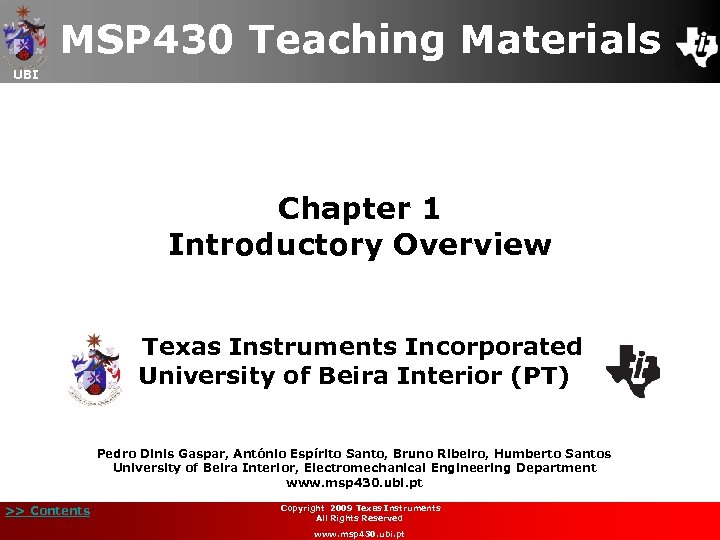MSP 430 Teaching Materials UBI Chapter 1 Introductory Overview Texas Instruments Incorporated University of Beira Interior (PT) Pedro Dinis Gaspar, António Espírito Santo, Bruno Ribeiro, Humberto Santos University of Beira Interior, Electromechanical Engineering Department www. msp 430. ubi. pt >> Contents Copyright 2009 Texas Instruments All Rights Reserved www. msp 430. ubi. ptContents UBI q Analogue and digital signals and systems q Mathematical notations q Floating and fixed-point arithmetic q How to read technical datasheets q Programming issues q Operators and expressions q Good software practices for low power consumption q Quiz >> Contents Copyright 2009 Texas Instruments All Rights Reserved www. msp 430. ubi. pt 2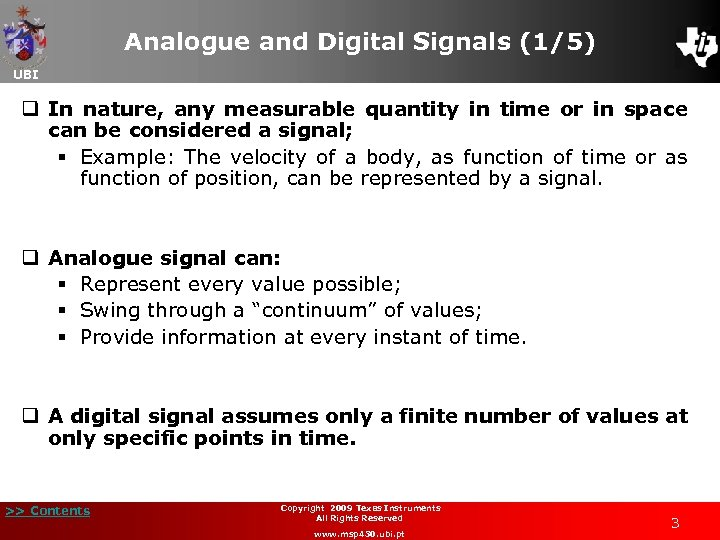Analogue and Digital Signals (1/5) UBI q In nature, any measurable quantity in time or in space can be considered a signal; § Example: The velocity of a body, as function of time or as function of position, can be represented by a signal. q Analogue signal can: § Represent every value possible; § Swing through a “continuum” of values; § Provide information at every instant of time. q A digital signal assumes only a finite number of values at only specific points in time. >> Contents Copyright 2009 Texas Instruments All Rights Reserved www. msp 430. ubi. pt 3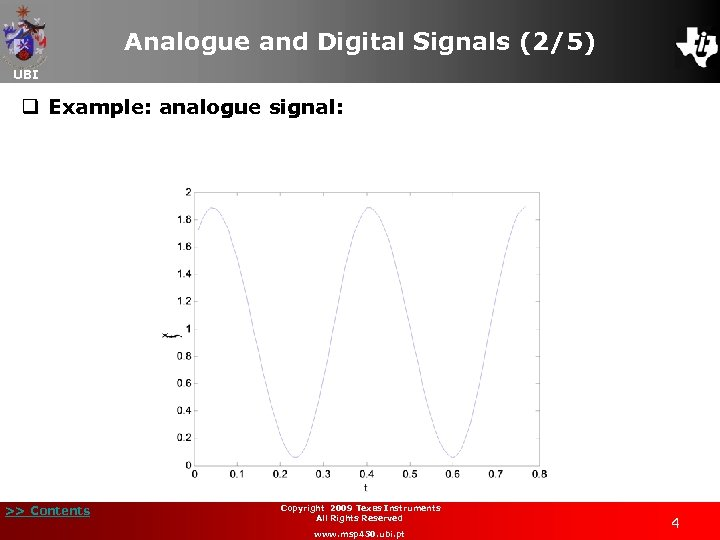Analogue and Digital Signals (2/5) UBI q Example: analogue signal: >> Contents Copyright 2009 Texas Instruments All Rights Reserved www. msp 430. ubi. pt 4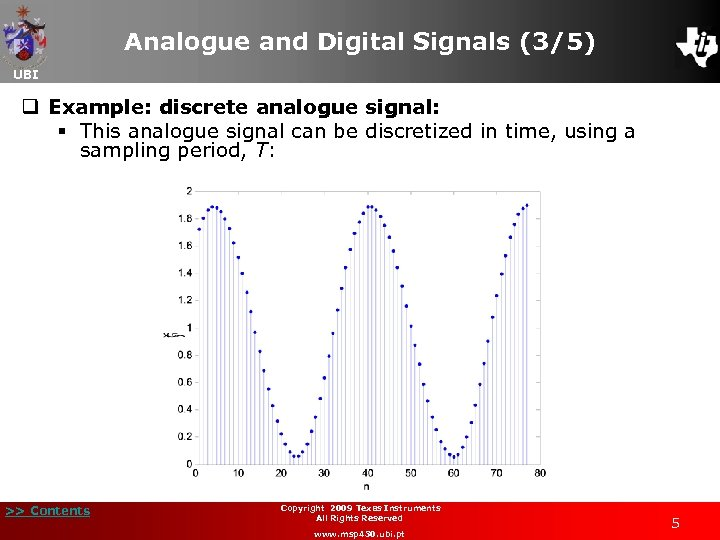Analogue and Digital Signals (3/5) UBI q Example: discrete analogue signal: § This analogue signal can be discretized in time, using a sampling period, T: >> Contents Copyright 2009 Texas Instruments All Rights Reserved www. msp 430. ubi. pt 5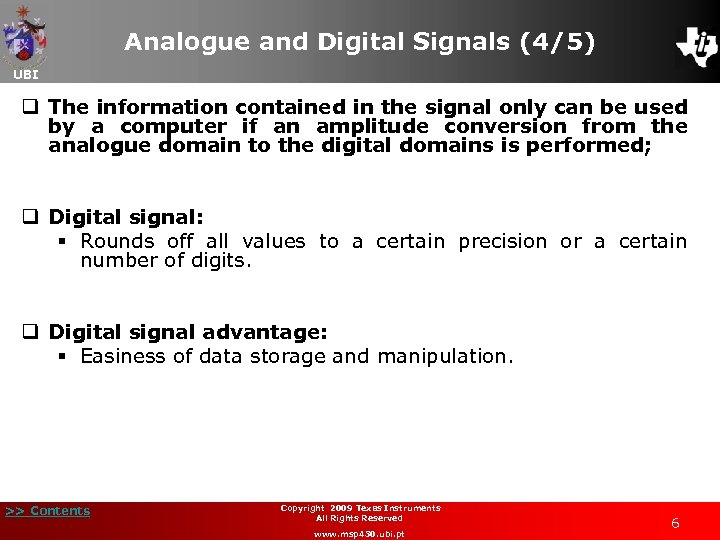Analogue and Digital Signals (4/5) UBI q The information contained in the signal only can be used by a computer if an amplitude conversion from the analogue domain to the digital domains is performed; q Digital signal: § Rounds off all values to a certain precision or a certain number of digits. q Digital signal advantage: § Easiness of data storage and manipulation. >> Contents Copyright 2009 Texas Instruments All Rights Reserved www. msp 430. ubi. pt 6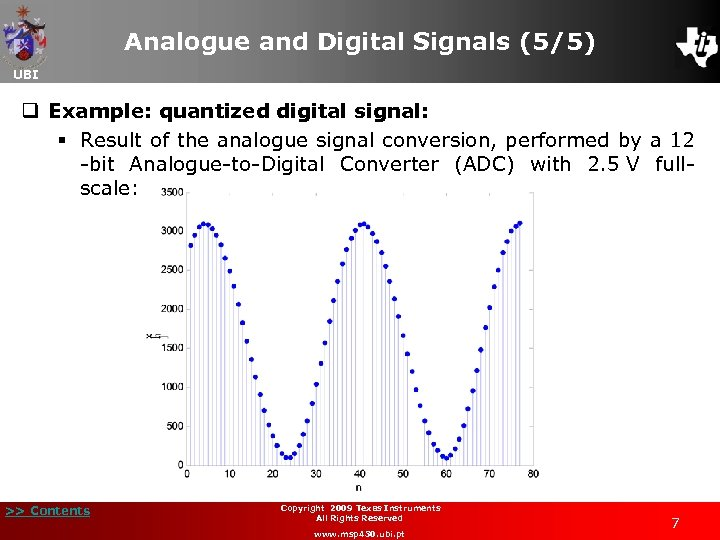Analogue and Digital Signals (5/5) UBI q Example: quantized digital signal: § Result of the analogue signal conversion, performed by a 12 -bit Analogue-to-Digital Converter (ADC) with 2. 5 V fullscale: >> Contents Copyright 2009 Texas Instruments All Rights Reserved www. msp 430. ubi. pt 7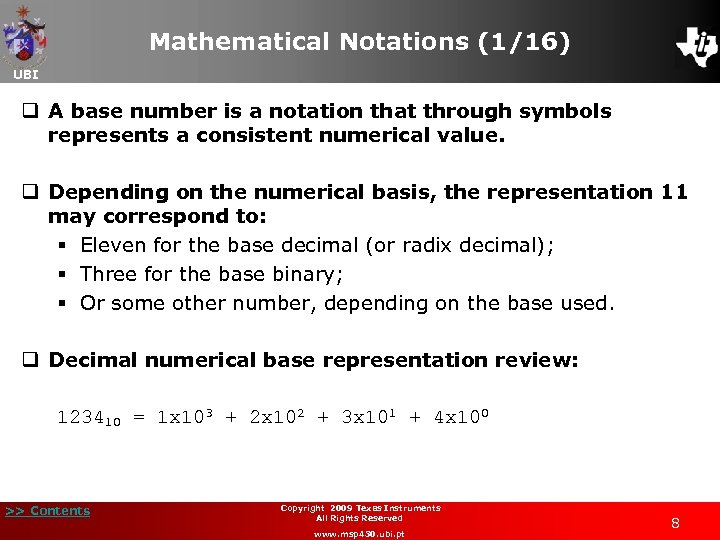Mathematical Notations (1/16) UBI q A base number is a notation that through symbols represents a consistent numerical value. q Depending on the numerical basis, the representation 11 may correspond to: § Eleven for the base decimal (or radix decimal); § Three for the base binary; § Or some other number, depending on the base used. q Decimal numerical base representation review: 123410 = 1 x 103 + 2 x 102 + 3 x 101 + 4 x 100 >> Contents Copyright 2009 Texas Instruments All Rights Reserved www. msp 430. ubi. pt 8Mathematical Notations (2/16) UBI q Decimal numerical base representation review: 123410 = 1 x 103 + 2 x 102 + 3 x 101 + 4 x 100 § Each of the symbols is multiplied by a weight of base 10; § As it moves from right to left the weight is increased. q General form for a number in base b: an-1 bn-1 + an-2 bn-2 +. . . + a 0 b 0 § n is the length of the numerical representation; § {an-1, an-2, …, a 0} is the numerical representation ordered increasingly (natural numbers of the set {0, …, b}). >> Contents Copyright 2009 Texas Instruments All Rights Reserved www. msp 430. ubi. pt 9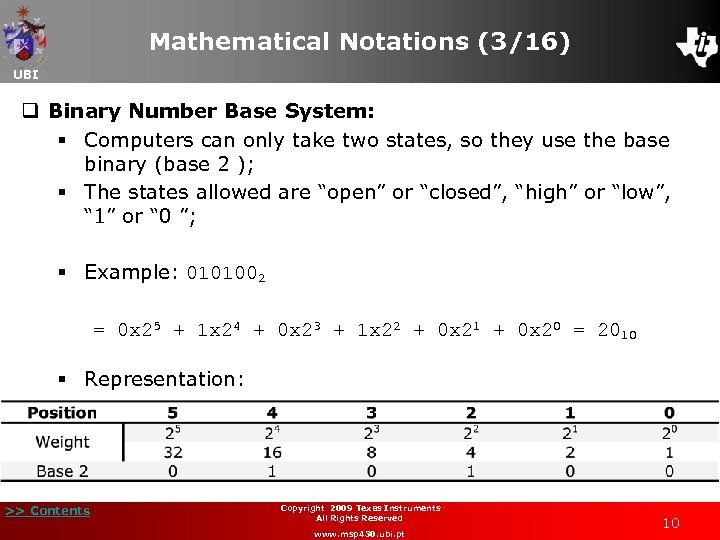Mathematical Notations (3/16) UBI q Binary Number Base System: § Computers can only take two states, so they use the base binary (base 2 ); § The states allowed are “open” or “closed”, “high” or “low”, “ 1” or “ 0 ”; § Example: 0101002 = 0 x 25 + 1 x 24 + 0 x 23 + 1 x 22 + 0 x 21 + 0 x 20 = 2010 § Representation: >> Contents Copyright 2009 Texas Instruments All Rights Reserved www. msp 430. ubi. pt 10Mathematical Notations (4/16) UBI q Hexadecimal Number Base System: § A difficulty that arises from the binary system is that it is verbose; § To represent the value 20210 in base 2 (binary) requires eight binary digits; § The decimal version requires only three decimal digits and so represents numbers much more compactly; § The hexadecimal (base 16) numbering system solves these problems. Hexadecimal numbers offer two useful features: • Hexadecimal numbers are very compact; • It is easy to convert from hexadecimal to binary and binary to hexadecimal. >> Contents Copyright 2009 Texas Instruments All Rights Reserved www. msp 430. ubi. pt 11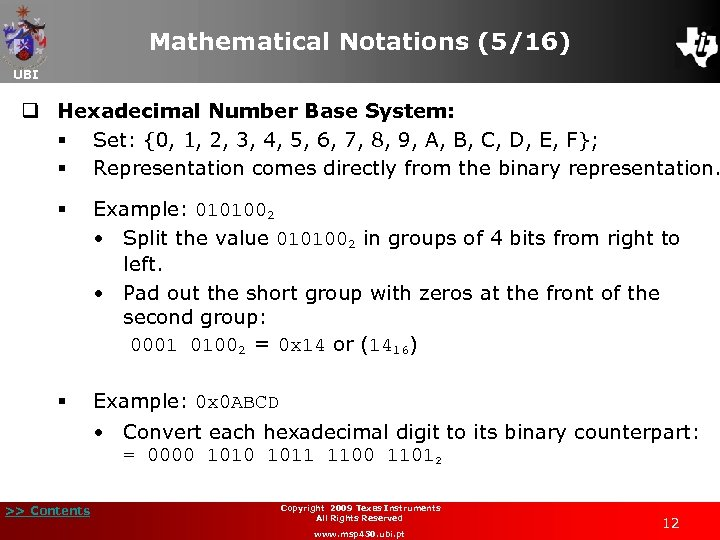Mathematical Notations (5/16) UBI q Hexadecimal Number Base System: § Set: {0, 1, 2, 3, 4, 5, 6, 7, 8, 9, A, B, C, D, E, F}; § Representation comes directly from the binary representation. § Example: 0101002 • Split the value 0101002 in groups of 4 bits from right to left. • Pad out the short group with zeros at the front of the second group: 0001 01002 = 0 x 14 or (1416) § Example: 0 x 0 ABCD • Convert each hexadecimal digit to its binary counterpart: = 0000 1011 1100 11012 >> Contents Copyright 2009 Texas Instruments All Rights Reserved www. msp 430. ubi. pt 12Mathematical Notations (6/16) UBI q Decimal to Binary Conversion: § Example 1: Decimal number 72110 to binary conversion: 72110 = 700 + 20 + 1 = 7 x 102 + 2 x 101 + 1 x 100 >> Contents 210 = 102410 (Higher than 3 rd power decimal value, 103); 29 = 51210 (MSB: 1 x 29): 721 - 512 = 209; 28 = 25610 (Higher than 209, the 2 nd bit: 0 x 28); 27 = 12810 (3 rd bit is: 1 x 27): 209 - 128 = 81; 26 = 6410 (4 th bit is: 1 x 26): 81 - 64 = 17; 25 = 3210 (Higher than 17, the 5 th bit is: 0 x 25); 24 = 1610 (6 th bit is: 1 x 24): 17 - 16 = 1; 23 = 810 (Higher than 1, the 7 th bit: 0 x 23) 22 = 410 (Higher than 1, the 8 th bit: 0 x 22); 21 = 210 (Higher than 1, the 9 th bit: 0 x 21); 20 = 110 (10 th bit - LSB): 1 x 20; Result: 72110 = 10110100012 Copyright 2009 Texas Instruments All Rights Reserved www. msp 430. ubi. pt 13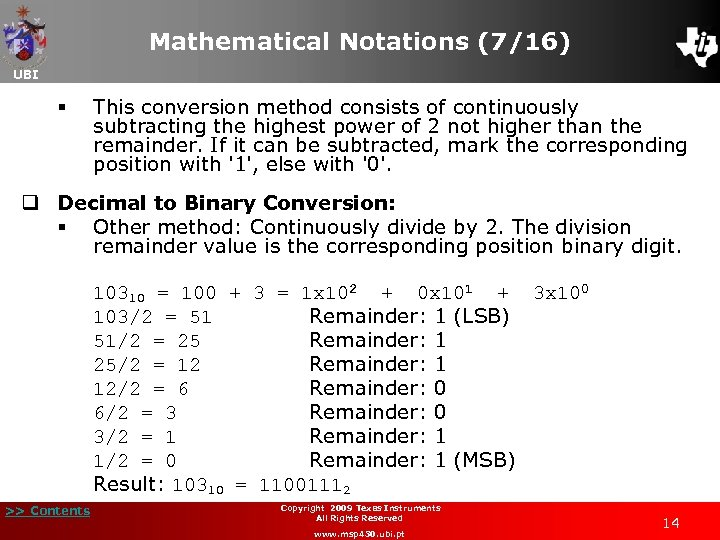Mathematical Notations (7/16) UBI § This conversion method consists of continuously subtracting the highest power of 2 not higher than the remainder. If it can be subtracted, mark the corresponding position with '1', else with '0'. q Decimal to Binary Conversion: § Other method: Continuously divide by 2. The division remainder value is the corresponding position binary digit. 10310 = 100 + 3 = 1 x 102 + 0 x 101 + 3 x 100 103/2 = 51 Remainder: 1 (LSB) 51/2 = 25 Remainder: 1 25/2 = 12 Remainder: 1 12/2 = 6 Remainder: 0 6/2 = 3 Remainder: 0 3/2 = 1 Remainder: 1 1/2 = 0 Remainder: 1 (MSB) Result: 10310 = 11001112 >> Contents Copyright 2009 Texas Instruments All Rights Reserved www. msp 430. ubi. pt 14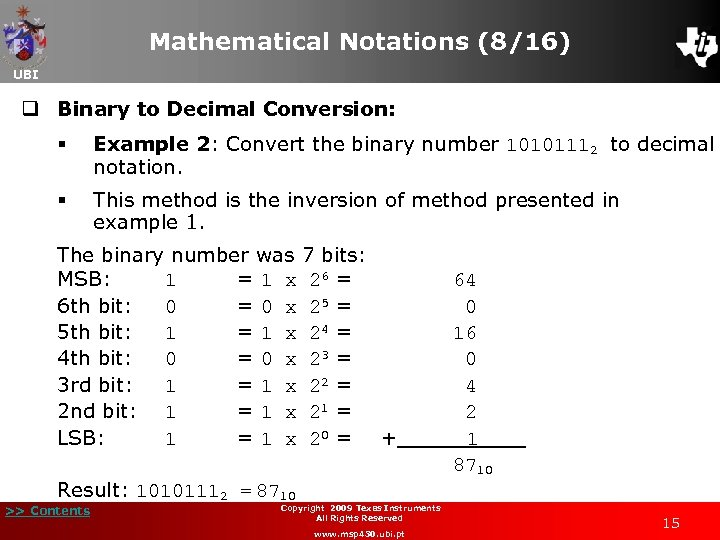Mathematical Notations (8/16) UBI q Binary to Decimal Conversion: § Example 2: Convert the binary number 10101112 to decimal notation. § This method is the inversion of method presented in example 1. The binary number was 7 bits: MSB: 1 = 1 x 26 = 6 th bit: 0 = 0 x 25 = 5 th bit: 1 = 1 x 24 = 4 th bit: 0 = 0 x 23 = 3 rd bit: 1 = 1 x 22 = 2 nd bit: 1 = 1 x 21 = LSB: 1 = 1 x 20 = + Result: 10101112 = 8710 >> Contents Copyright 2009 Texas Instruments All Rights Reserved www. msp 430. ubi. pt 64 0 16 0 4 2 1 8710 15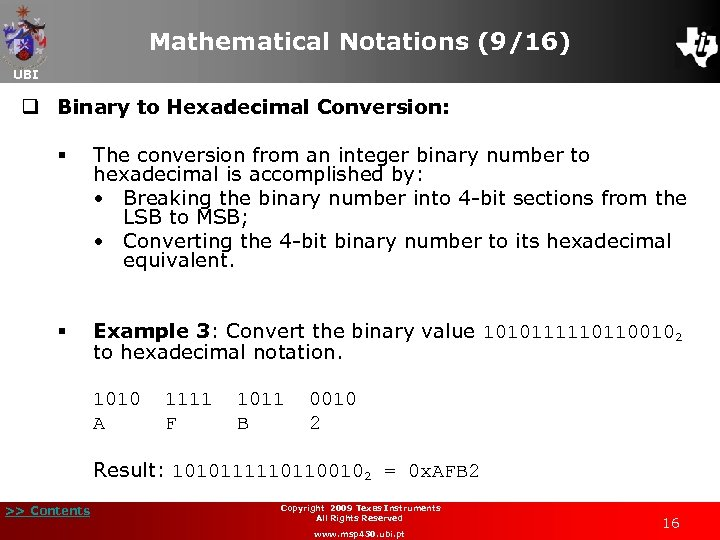Mathematical Notations (9/16) UBI q Binary to Hexadecimal Conversion: § The conversion from an integer binary number to hexadecimal is accomplished by: • Breaking the binary number into 4 -bit sections from the LSB to MSB; • Converting the 4 -bit binary number to its hexadecimal equivalent. § Example 3: Convert the binary value 10101111101100102 to hexadecimal notation. 1010 1111 1011 0010 A F B 2 Result: 10101111101100102 = 0 x. AFB 2 >> Contents Copyright 2009 Texas Instruments All Rights Reserved www. msp 430. ubi. pt 16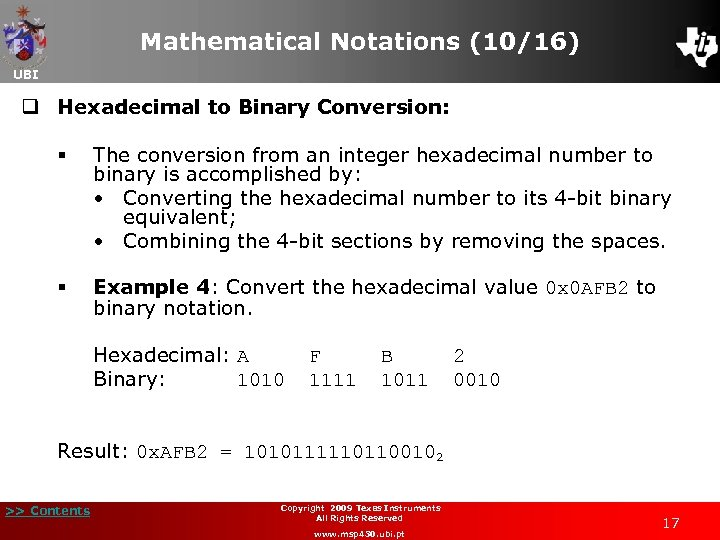Mathematical Notations (10/16) UBI q Hexadecimal to Binary Conversion: § The conversion from an integer hexadecimal number to binary is accomplished by: • Converting the hexadecimal number to its 4 -bit binary equivalent; • Combining the 4 -bit sections by removing the spaces. § Example 4: Convert the hexadecimal value 0 x 0 AFB 2 to binary notation. Hexadecimal: A Binary: 1010 F B 2 1111 1011 0010 Result: 0 x. AFB 2 = 10101111101100102 >> Contents Copyright 2009 Texas Instruments All Rights Reserved www. msp 430. ubi. pt 17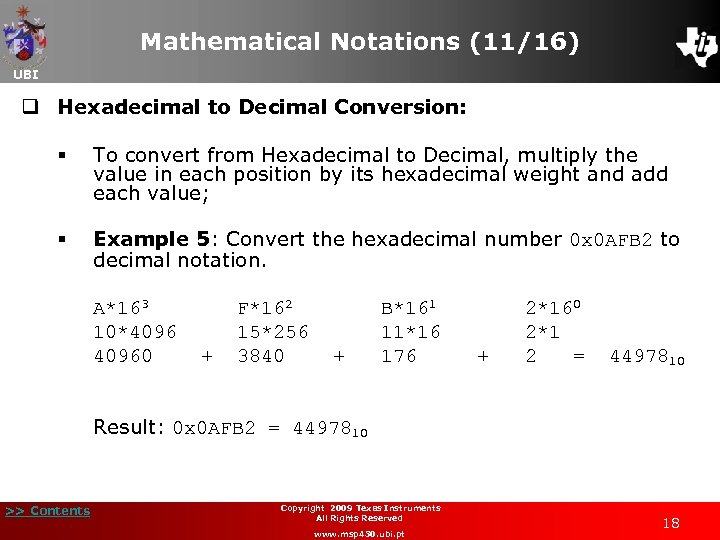Mathematical Notations (11/16) UBI q Hexadecimal to Decimal Conversion: § To convert from Hexadecimal to Decimal, multiply the value in each position by its hexadecimal weight and add each value; § Example 5: Convert the hexadecimal number 0 x 0 AFB 2 to decimal notation. A*163 F*162 B*161 2*160 10*4096 15*256 11*16 2*1 40960 + 3840 + 176 + 2 = 4497810 Result: 0 x 0 AFB 2 = 4497810 >> Contents Copyright 2009 Texas Instruments All Rights Reserved www. msp 430. ubi. pt 18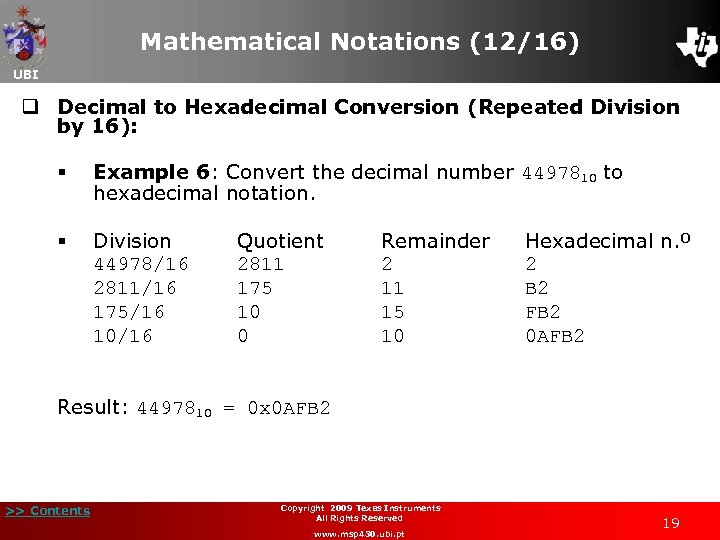Mathematical Notations (12/16) UBI q Decimal to Hexadecimal Conversion (Repeated Division by 16): § Example 6: Convert the decimal number 4497810 to hexadecimal notation. § Division 44978/16 2811/16 175/16 10/16 Quotient 2811 175 10 0 Remainder 2 11 15 10 Hexadecimal n. º 2 B 2 FB 2 0 AFB 2 Result: 4497810 = 0 x 0 AFB 2 >> Contents Copyright 2009 Texas Instruments All Rights Reserved www. msp 430. ubi. pt 19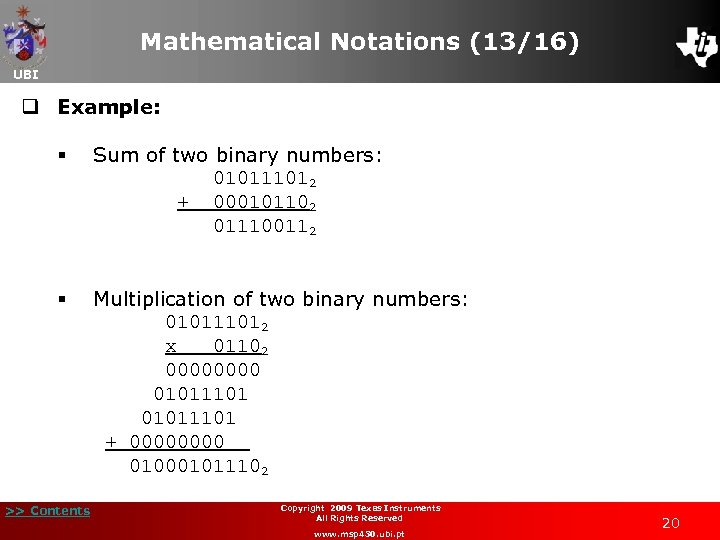Mathematical Notations (13/16) UBI q Example: Sum of two binary numbers: 010111012 + 000101102 011100112 § § >> Contents Multiplication of two binary numbers: 010111012 x 01102 0000 01011101 + 0000 010001011102 Copyright 2009 Texas Instruments All Rights Reserved www. msp 430. ubi. pt 20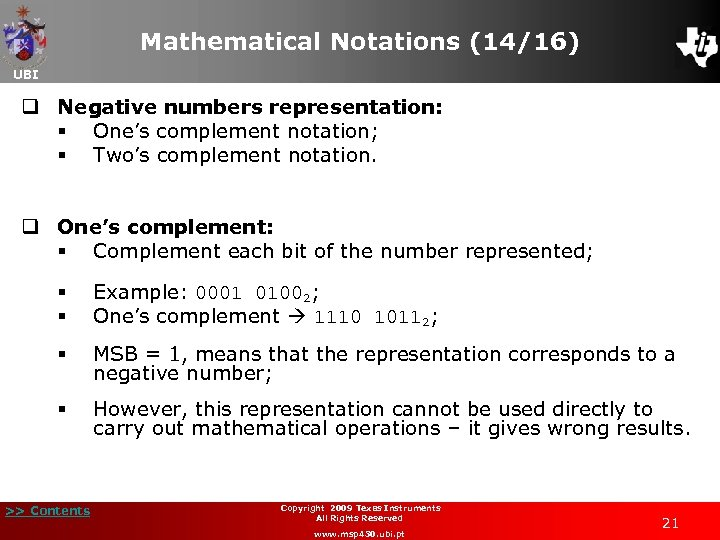Mathematical Notations (14/16) UBI q Negative numbers representation: § One’s complement notation; § Two’s complement notation. q One’s complement: § Complement each bit of the number represented; § § Example: 0001 01002; One’s complement 1110 10112; § MSB = 1, means that the representation corresponds to a negative number; § However, this representation cannot be used directly to carry out mathematical operations – it gives wrong results. >> Contents Copyright 2009 Texas Instruments All Rights Reserved www. msp 430. ubi. pt 21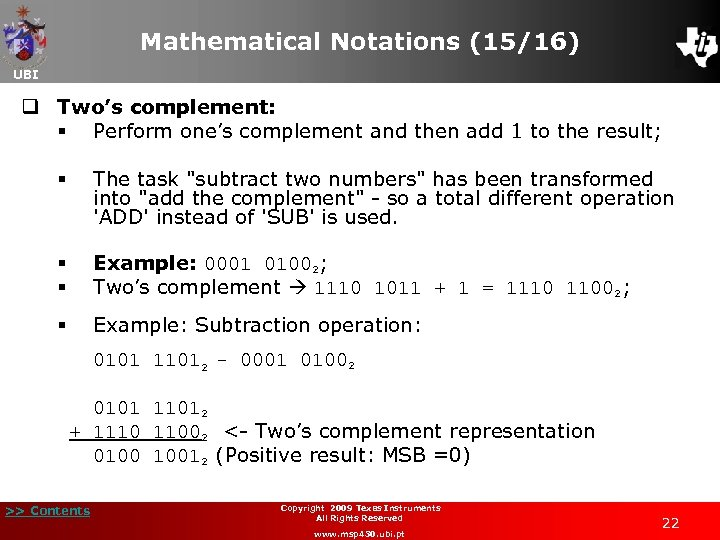Mathematical Notations (15/16) UBI q Two’s complement: § Perform one’s complement and then add 1 to the result; § § § § The task "subtract two numbers" has been transformed into "add the complement" - so a total different operation 'ADD' instead of 'SUB' is used. Example: 0001 01002; Two’s complement 1110 1011 + 1 = 1110 11002; Example: Subtraction operation: 0101 11012 - 0001 01002 0101 11012 + 1110 11002 <- Two’s complement representation 0100 10012 (Positive result: MSB =0) >> Contents Copyright 2009 Texas Instruments All Rights Reserved www. msp 430. ubi. pt 22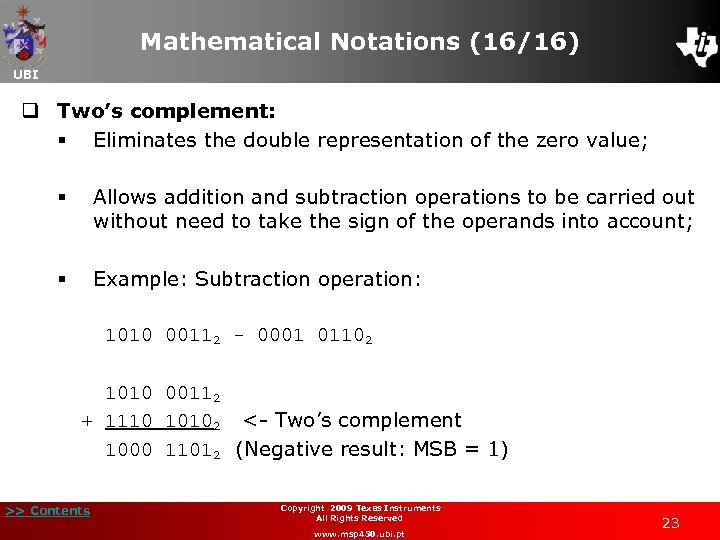Mathematical Notations (16/16) UBI q Two’s complement: § Eliminates the double representation of the zero value; § Allows addition and subtraction operations to be carried out without need to take the sign of the operands into account; § Example: Subtraction operation: 1010 00112 - 0001 01102 1010 00112 + 1110 10102 <- Two’s complement 1000 11012 (Negative result: MSB = 1) >> Contents Copyright 2009 Texas Instruments All Rights Reserved www. msp 430. ubi. pt 23Floating and Fixed-point Arithmetic (1/15) UBI q Representation of fractional numbers: q Two different ways: § Fixed-point; § Floating-point. q Fixed-point representation: § Allows us to represent the fractional part; § Consumes less processing resources than floating-point; >> Contents Copyright 2009 Texas Instruments All Rights Reserved www. msp 430. ubi. pt 24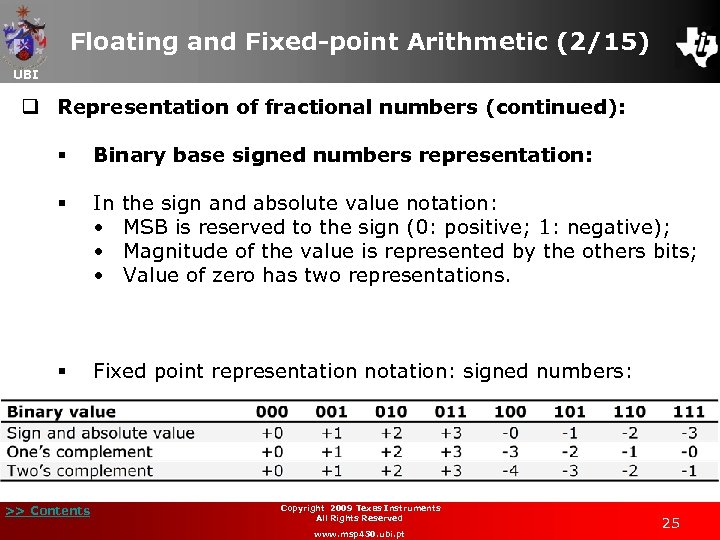Floating and Fixed-point Arithmetic (2/15) UBI q Representation of fractional numbers (continued): § Binary base signed numbers representation: § In the sign and absolute value notation: • MSB is reserved to the sign (0: positive; 1: negative); • Magnitude of the value is represented by the others bits; • Value of zero has two representations. § Fixed point representation notation: signed numbers: >> Contents Copyright 2009 Texas Instruments All Rights Reserved www. msp 430. ubi. pt 25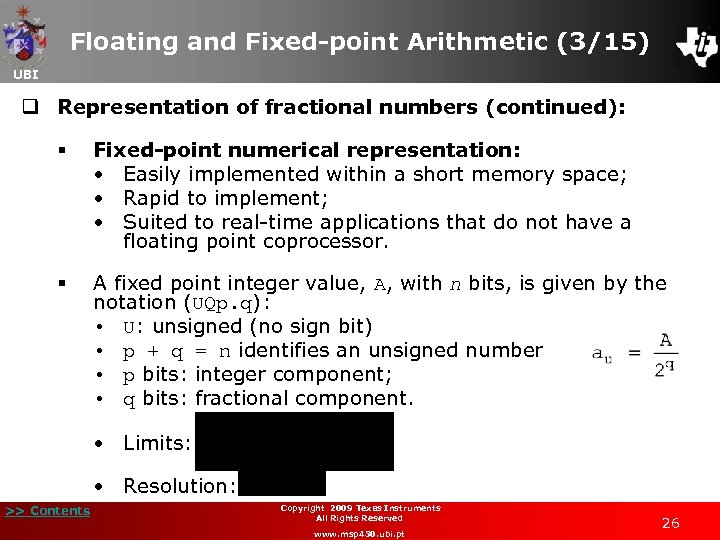Floating and Fixed-point Arithmetic (3/15) UBI q Representation of fractional numbers (continued): § Fixed-point numerical representation: • Easily implemented within a short memory space; • Rapid to implement; • Suited to real-time applications that do not have a floating point coprocessor. § A fixed point integer value, A, with n bits, is given by the notation (UQp. q): • U: unsigned (no sign bit) • p + q = n identifies an unsigned number • p bits: integer component; • q bits: fractional component. • Limits: • Resolution: >> Contents Copyright 2009 Texas Instruments All Rights Reserved www. msp 430. ubi. pt 26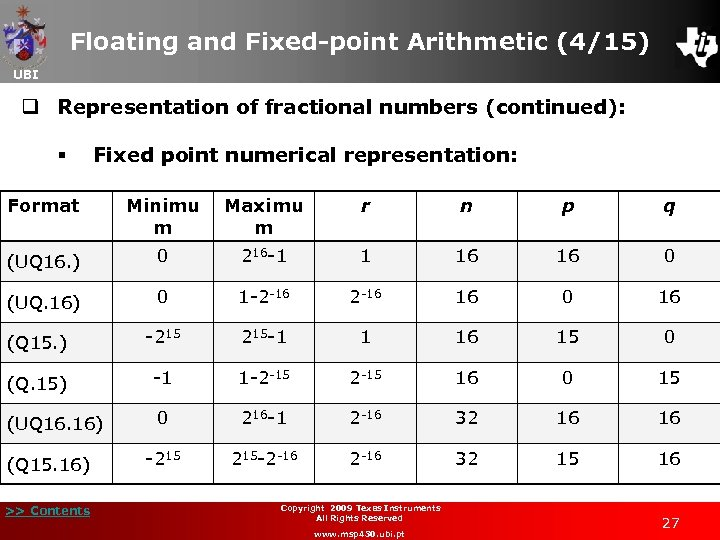Floating and Fixed-point Arithmetic (4/15) UBI q Representation of fractional numbers (continued): § Fixed point numerical representation: Format Minimu m Maximu m r n p q (UQ 16. ) 0 216 -1 1 16 16 0 (UQ. 16) 0 1 -2 -16 16 0 16 (Q 15. ) -215 215 -1 1 16 15 0 (Q. 15) -1 1 -2 -15 16 0 15 (UQ 16. 16) 0 216 -1 2 -16 32 16 16 -215 215 -2 -16 32 15 16 (Q 15. 16) >> Contents Copyright 2009 Texas Instruments All Rights Reserved www. msp 430. ubi. pt 27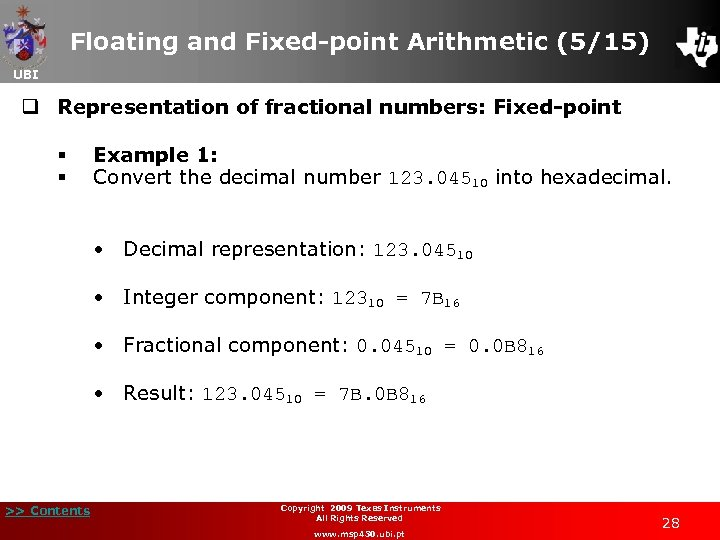Floating and Fixed-point Arithmetic (5/15) UBI q Representation of fractional numbers: Fixed-point § § Example 1: Convert the decimal number 123. 04510 into hexadecimal. • Decimal representation: 123. 04510 • Integer component: 12310 = 7 B 16 • Fractional component: 0. 04510 = 0. 0 B 816 • Result: 123. 04510 = 7 B. 0 B 816 >> Contents Copyright 2009 Texas Instruments All Rights Reserved www. msp 430. ubi. pt 28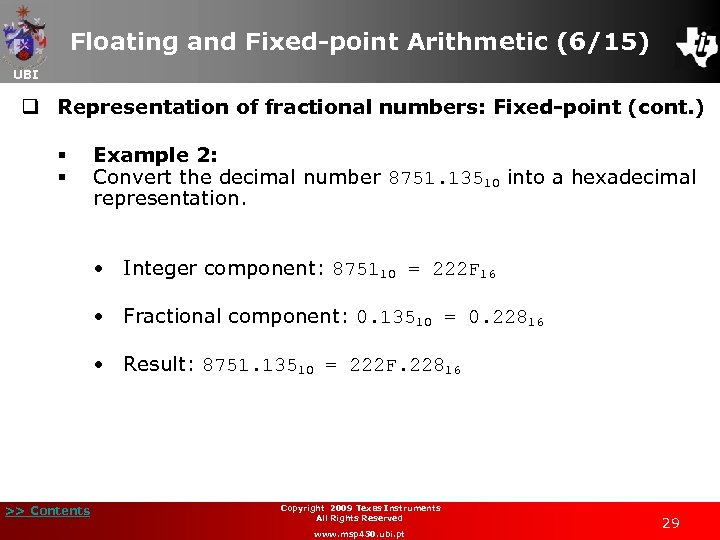Floating and Fixed-point Arithmetic (6/15) UBI q Representation of fractional numbers: Fixed-point (cont. ) § § Example 2: Convert the decimal number 8751. 13510 into a hexadecimal representation. • Integer component: 875110 = 222 F 16 • Fractional component: 0. 13510 = 0. 22816 • Result: 8751. 13510 = 222 F. 22816 >> Contents Copyright 2009 Texas Instruments All Rights Reserved www. msp 430. ubi. pt 29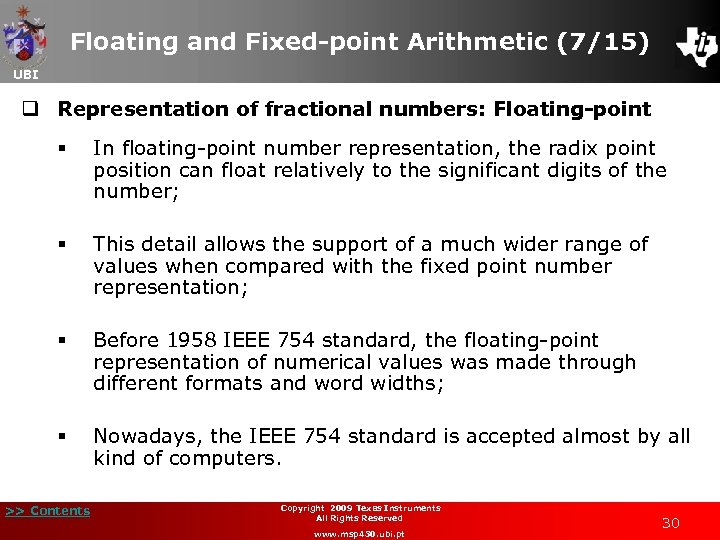Floating and Fixed-point Arithmetic (7/15) UBI q Representation of fractional numbers: Floating-point § In floating-point number representation, the radix point position can float relatively to the significant digits of the number; § This detail allows the support of a much wider range of values when compared with the fixed point number representation; § Before 1958 IEEE 754 standard, the floating-point representation of numerical values was made through different formats and word widths; § Nowadays, the IEEE 754 standard is accepted almost by all kind of computers. >> Contents Copyright 2009 Texas Instruments All Rights Reserved www. msp 430. ubi. pt 30Floating and Fixed-point Arithmetic (8/15) UBI q Representation of fractional numbers: Floating-point (cont. ) § From the set of formats supported by IEEE 754 standard, the most widely used are the single-precision (32 -bit) and double-precision (64 -bit); § Moreover, the standard also establishes others kinds of formats: "quad" binary and decimal floating-point (128 -bit); and double decimal floating-point (64 -bit); § Less used are the extended precision (80 -bit) and halfprecision (16 -bit) formats. The last one appears in the IEEE 754 r proposed revision; § Standard current version is IEEE 754 -2008, which was published in August 2008. >> Contents Copyright 2008 Texas Instruments 2009 All Rights Reserved www. msp 430. ubi. pt 31Floating and Fixed-point Arithmetic (9/15) UBI q Representation of fractional numbers: Floating-point (cont. ) § In the data structure of floating-point numbers, the most significant bit is the sign bit (S), followed by the exponent that is biased; § The mantissa without the most significant bit is stored after the exponent in the fraction field: § The exponent is biased by the (2 e − 1) − 1, where e is the exponent number of bits; § This solution was adopted because exponents must be signed, and the two's complement representation will require extra computational work if it was used. >> Contents Copyright 2008 Texas Instruments 2009 All Rights Reserved www. msp 430. ubi. pt 32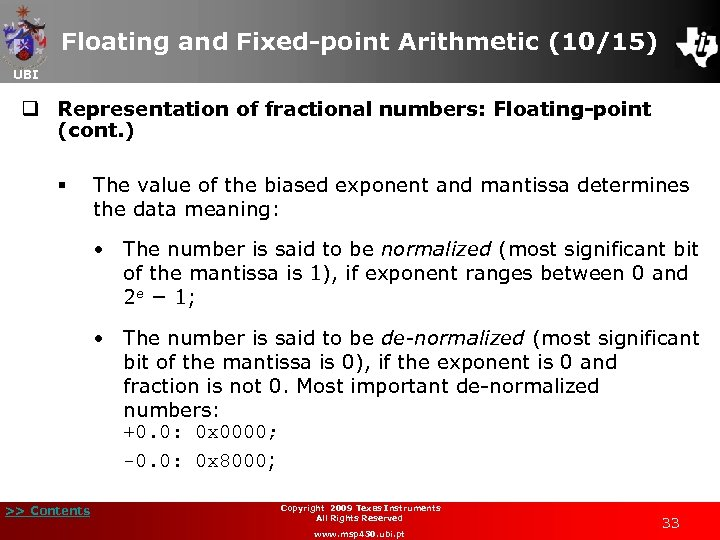Floating and Fixed-point Arithmetic (10/15) UBI q Representation of fractional numbers: Floating-point (cont. ) § The value of the biased exponent and mantissa determines the data meaning: • The number is said to be normalized (most significant bit of the mantissa is 1), if exponent ranges between 0 and 2 e − 1; • The number is said to be de-normalized (most significant bit of the mantissa is 0), if the exponent is 0 and fraction is not 0. Most important de-normalized numbers: +0. 0: 0 x 0000; -0. 0: 0 x 8000; >> Contents Copyright 2009 Texas Instruments All Rights Reserved www. msp 430. ubi. pt 33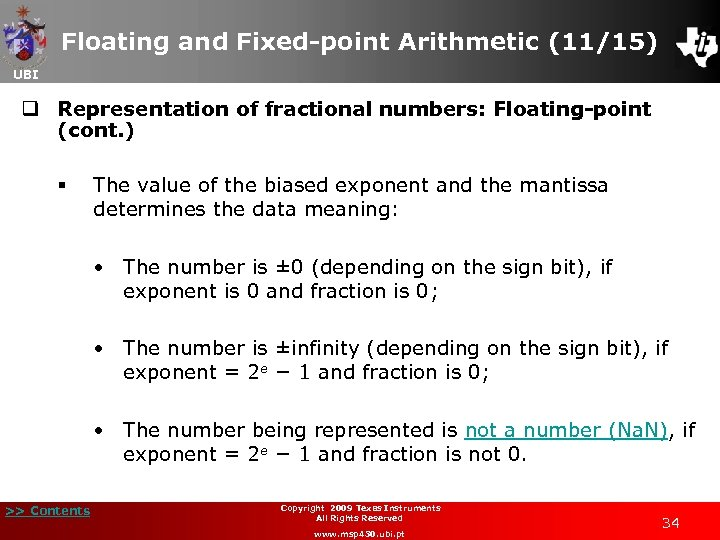Floating and Fixed-point Arithmetic (11/15) UBI q Representation of fractional numbers: Floating-point (cont. ) § The value of the biased exponent and the mantissa determines the data meaning: • The number is ± 0 (depending on the sign bit), if exponent is 0 and fraction is 0; • The number is ±infinity (depending on the sign bit), if exponent = 2 e − 1 and fraction is 0; • The number being represented is not a number (Na. N), if exponent = 2 e − 1 and fraction is not 0. >> Contents Copyright 2009 Texas Instruments All Rights Reserved www. msp 430. ubi. pt 34Floating and Fixed-point Arithmetic (12/15) UBI q Representation of fractional numbers: Floating-point (cont. ) § Format The IEEE 754 binary formats have the following organization: Sign Exponent bias Mantissa Total number of bits Half 1 5 15 10 16 Single 1 8 127 23 32 Double 1 11 1023 52 64 Quad 1 15 16383 112 128 § A number X has the value: X = >> Contents Copyright 2009 Texas Instruments All Rights Reserved www. msp 430. ubi. pt 35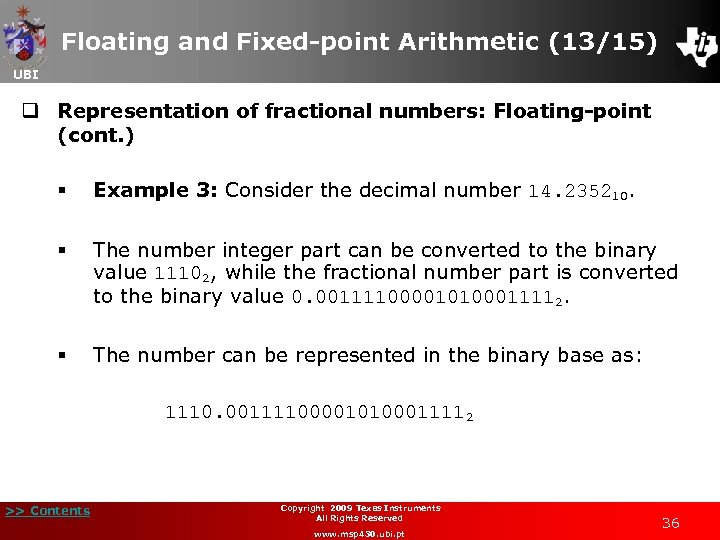Floating and Fixed-point Arithmetic (13/15) UBI q Representation of fractional numbers: Floating-point (cont. ) § Example 3: Consider the decimal number 14. 235210. § The number integer part can be converted to the binary value 11102, while the fractional number part is converted to the binary value 0. 001111000010100011112. § The number can be represented in the binary base as: 1110. 001111000010100011112 >> Contents Copyright 2009 Texas Instruments All Rights Reserved www. msp 430. ubi. pt 36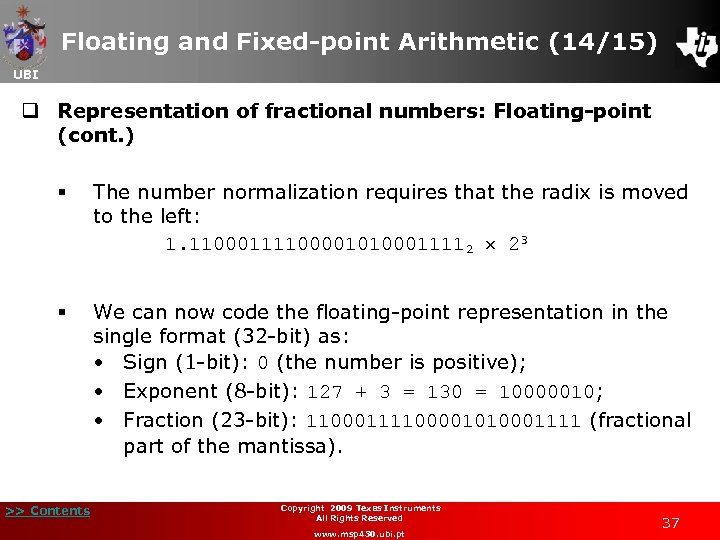Floating and Fixed-point Arithmetic (14/15) UBI q Representation of fractional numbers: Floating-point (cont. ) § The number normalization requires that the radix is moved to the left: 1. 110001111000010100011112 23 § We can now code the floating-point representation in the single format (32 -bit) as: • Sign (1 -bit): 0 (the number is positive); • Exponent (8 -bit): 127 + 3 = 130 = 10000010; • Fraction (23 -bit): 11000111100001010001111 (fractional part of the mantissa). >> Contents Copyright 2009 Texas Instruments All Rights Reserved www. msp 430. ubi. pt 37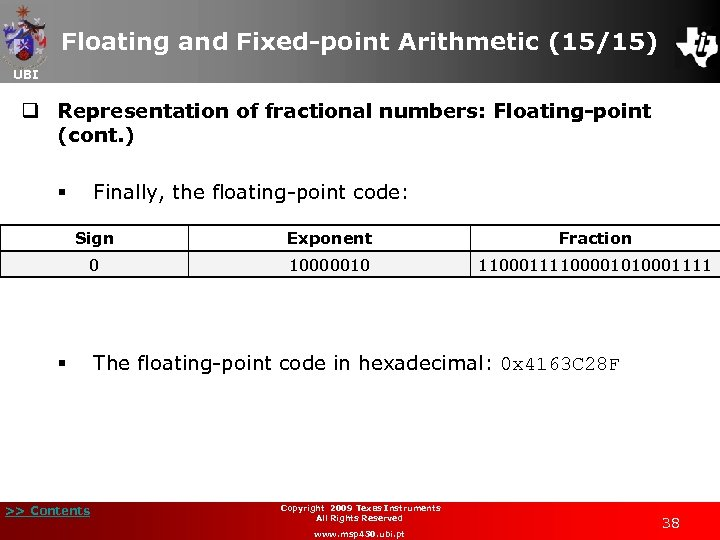Floating and Fixed-point Arithmetic (15/15) UBI q Representation of fractional numbers: Floating-point (cont. ) Finally, the floating-point code: § Sign Exponent Fraction 0 10000010 11000111100001010001111 § >> Contents The floating-point code in hexadecimal: 0 x 4163 C 28 F Copyright 2009 Texas Instruments All Rights Reserved www. msp 430. ubi. pt 38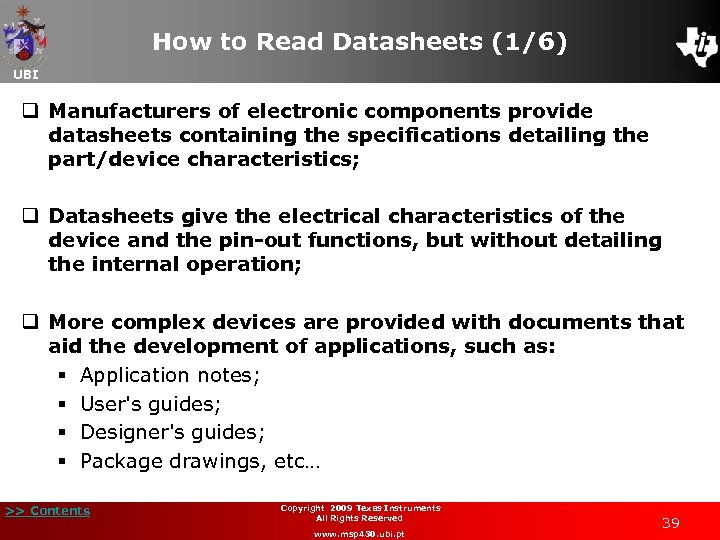How to Read Datasheets (1/6) UBI q Manufacturers of electronic components provide datasheets containing the specifications detailing the part/device characteristics; q Datasheets give the electrical characteristics of the device and the pin-out functions, but without detailing the internal operation; q More complex devices are provided with documents that aid the development of applications, such as: § Application notes; § User's guides; § Designer's guides; § Package drawings, etc… >> Contents Copyright 2009 Texas Instruments All Rights Reserved www. msp 430. ubi. pt 39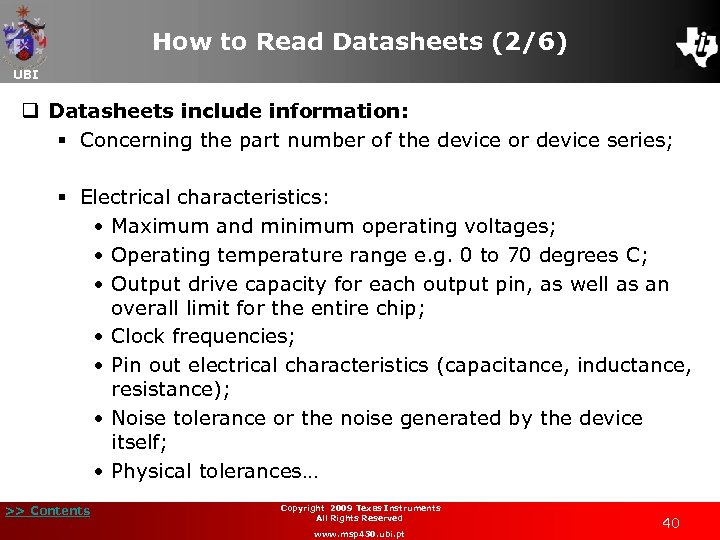How to Read Datasheets (2/6) UBI q Datasheets include information: § Concerning the part number of the device or device series; § Electrical characteristics: • Maximum and minimum operating voltages; • Operating temperature range e. g. 0 to 70 degrees C; • Output drive capacity for each output pin, as well as an overall limit for the entire chip; • Clock frequencies; • Pin out electrical characteristics (capacitance, inductance, resistance); • Noise tolerance or the noise generated by the device itself; • Physical tolerances… >> Contents Copyright 2009 Texas Instruments All Rights Reserved www. msp 430. ubi. pt 40How to Read Datasheets (3/6) UBI q MSP 430 device datasheet: § Device has a large number of peripherals; § Each input/output pin usually has more than one function; § It has a table with the description of each pin function; § Example, Pin number 2 = P 6. 3/A 3; • Digital Input/Output Port 6 bit 3; • 3 rd analogue input. >> Contents Copyright 2009 Texas Instruments All Rights Reserved www. msp 430. ubi. pt 41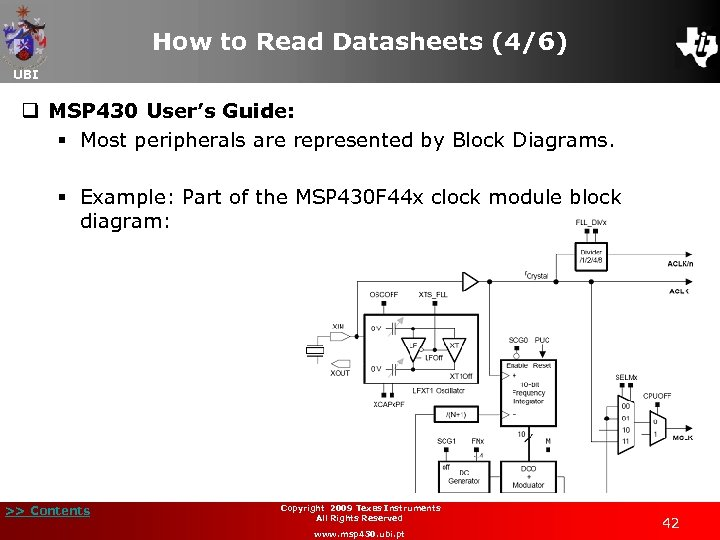How to Read Datasheets (4/6) UBI q MSP 430 User’s Guide: § Most peripherals are represented by Block Diagrams. § Example: Part of the MSP 430 F 44 x clock module block diagram: >> Contents Copyright 2009 Texas Instruments All Rights Reserved www. msp 430. ubi. pt 42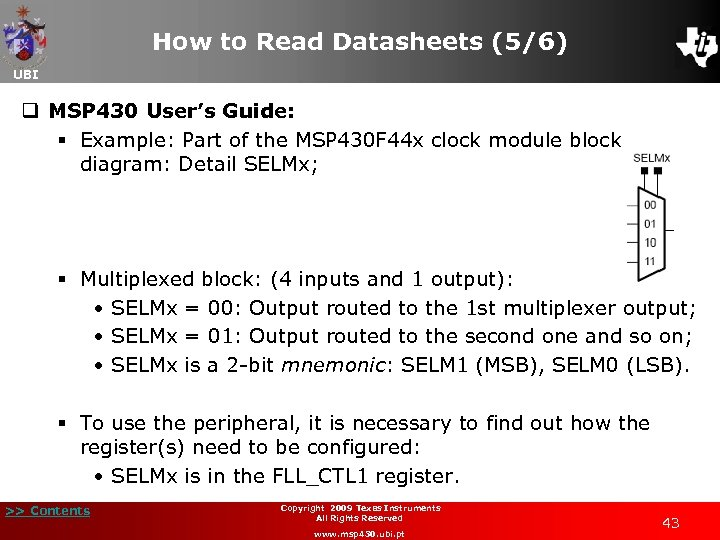How to Read Datasheets (5/6) UBI q MSP 430 User’s Guide: § Example: Part of the MSP 430 F 44 x clock module block diagram: Detail SELMx; § Multiplexed block: (4 inputs and 1 output): • SELMx = 00: Output routed to the 1 st multiplexer output; • SELMx = 01: Output routed to the second one and so on; • SELMx is a 2 -bit mnemonic: SELM 1 (MSB), SELM 0 (LSB). § To use the peripheral, it is necessary to find out how the register(s) need to be configured: • SELMx is in the FLL_CTL 1 register. >> Contents Copyright 2009 Texas Instruments All Rights Reserved www. msp 430. ubi. pt 43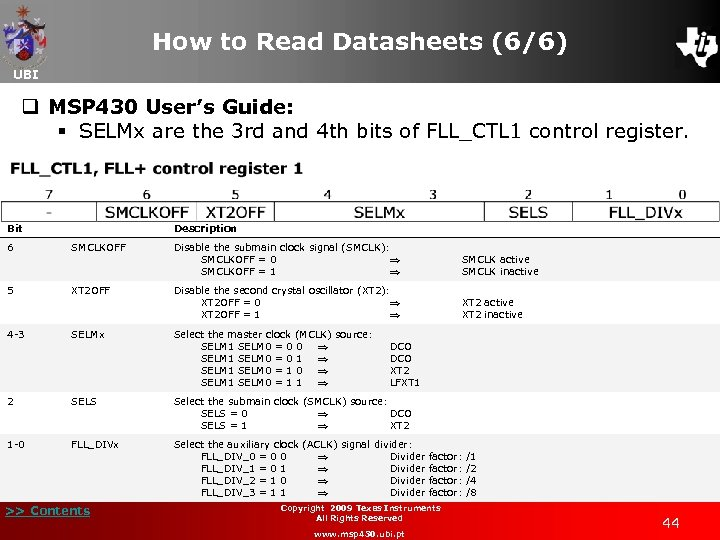How to Read Datasheets (6/6) UBI q MSP 430 User’s Guide: § SELMx are the 3 rd and 4 th bits of FLL_CTL 1 control register. Bit Description 6 SMCLKOFF Disable the submain clock signal (SMCLK): SMCLKOFF = 0 SMCLKOFF = 1 SMCLK active SMCLK inactive 5 XT 2 OFF Disable the second crystal oscillator (XT 2): XT 2 OFF = 0 XT 2 OFF = 1 XT 2 active XT 2 inactive 4 -3 SELMx Select the master clock (MCLK) source: SELM 1 SELM 0 = 0 0 SELM 1 SELM 0 = 0 1 SELM 1 SELM 0 = 1 0 SELM 1 SELM 0 = 1 1 2 SELS Select the submain clock (SMCLK) source: SELS = 0 DCO SELS = 1 XT 2 1 -0 FLL_DIVx Select the auxiliary clock (ACLK) signal divider: FLL_DIV_0 = 0 0 Divider factor: /1 FLL_DIV_1 = 0 1 Divider factor: /2 FLL_DIV_2 = 1 0 Divider factor: /4 FLL_DIV_3 = 1 1 Divider factor: /8 >> Contents DCO XT 2 LFXT 1 Copyright 2009 Texas Instruments All Rights Reserved www. msp 430. ubi. pt 44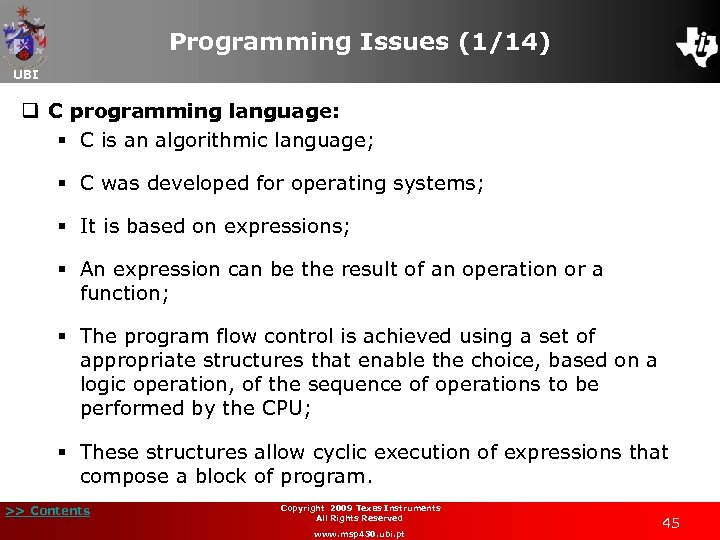Programming Issues (1/14) UBI q C programming language: § C is an algorithmic language; § C was developed for operating systems; § It is based on expressions; § An expression can be the result of an operation or a function; § The program flow control is achieved using a set of appropriate structures that enable the choice, based on a logic operation, of the sequence of operations to be performed by the CPU; § These structures allow cyclic execution of expressions that compose a block of program. >> Contents Copyright 2009 Texas Instruments All Rights Reserved www. msp 430. ubi. pt 45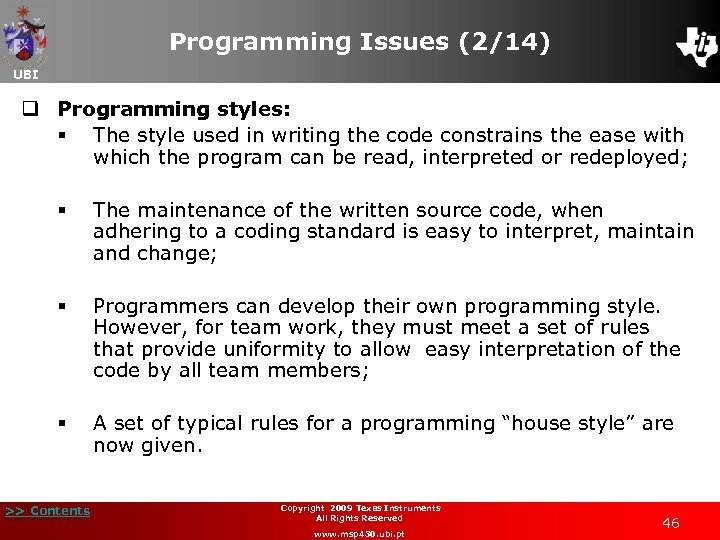Programming Issues (2/14) UBI q Programming styles: § The style used in writing the code constrains the ease with which the program can be read, interpreted or redeployed; § The maintenance of the written source code, when adhering to a coding standard is easy to interpret, maintain and change; § Programmers can develop their own programming style. However, for team work, they must meet a set of rules that provide uniformity to allow easy interpretation of the code by all team members; § A set of typical rules for a programming “house style” are now given. >> Contents Copyright 2009 Texas Instruments All Rights Reserved www. msp 430. ubi. pt 46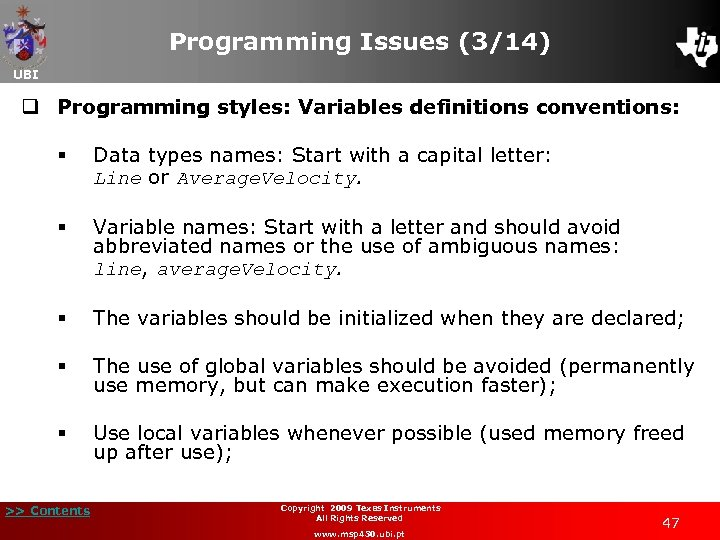Programming Issues (3/14) UBI q Programming styles: Variables definitions conventions: § Data types names: Start with a capital letter: Line or Average. Velocity. § Variable names: Start with a letter and should avoid abbreviated names or the use of ambiguous names: line, average. Velocity. § The variables should be initialized when they are declared; § The use of global variables should be avoided (permanently use memory, but can make execution faster); § Use local variables whenever possible (used memory freed up after use); >> Contents Copyright 2009 Texas Instruments All Rights Reserved www. msp 430. ubi. pt 47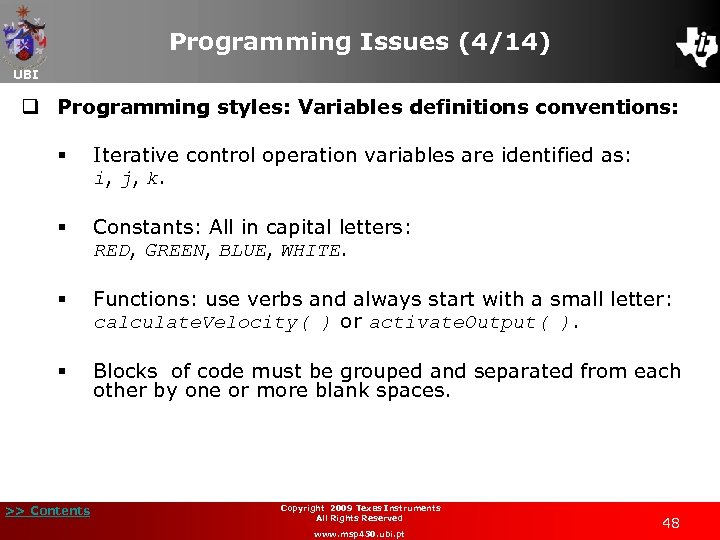Programming Issues (4/14) UBI q Programming styles: Variables definitions conventions: § Iterative control operation variables are identified as: i, j, k. § Constants: All in capital letters: RED, GREEN, BLUE, WHITE. § Functions: use verbs and always start with a small letter: calculate. Velocity( ) or activate. Output( ). § Blocks of code must be grouped and separated from each other by one or more blank spaces. >> Contents Copyright 2009 Texas Instruments All Rights Reserved www. msp 430. ubi. pt 48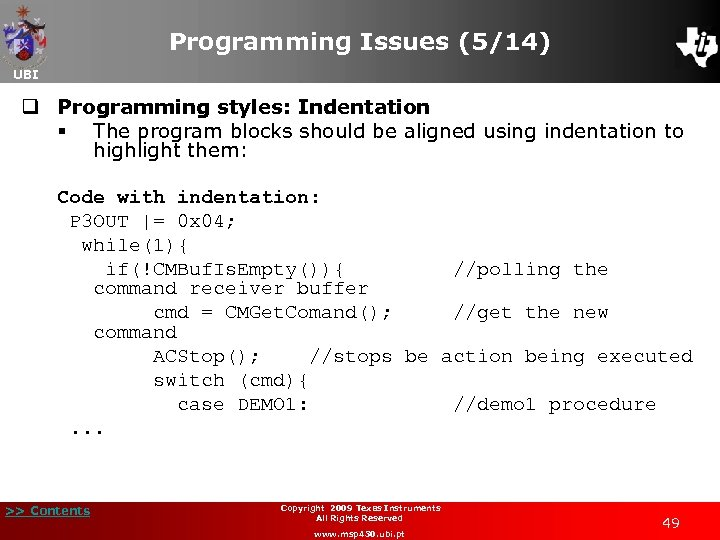Programming Issues (5/14) UBI q Programming styles: Indentation § The program blocks should be aligned using indentation to highlight them: Code with indentation: P 3 OUT |= 0 x 04; while(1){ if(!CMBuf. Is. Empty()){ //polling the command receiver buffer cmd = CMGet. Comand(); //get the new command ACStop(); //stops be action being executed switch (cmd){ case DEMO 1: //demo 1 procedure . . . >> Contents Copyright 2009 Texas Instruments All Rights Reserved www. msp 430. ubi. pt 49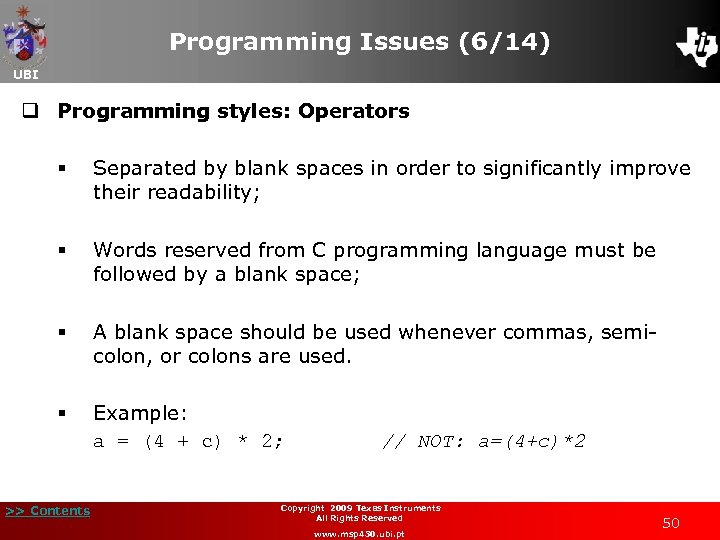Programming Issues (6/14) UBI q Programming styles: Operators § Separated by blank spaces in order to significantly improve their readability; § Words reserved from C programming language must be followed by a blank space; § A blank space should be used whenever commas, semicolon, or colons are used. § Example: a = (4 + c) * 2; // NOT: a=(4+c)*2 >> Contents Copyright 2009 Texas Instruments All Rights Reserved www. msp 430. ubi. pt 50Programming Issues (7/14) UBI q Programming styles: Comments § Source code should have additional information provided in the form of comments so it can be clearly interpreted; § Comment in a code line: Double diagonal ( // ); § Block of comments: Starts with ( /* ) and ends with ( */ ); § Example: /* The source code should be written in such a way that enough information is provided for the reader to fully understand the function of the code */ >> Contents Copyright 2009 Texas Instruments All Rights Reserved www. msp 430. ubi. pt 51Programming Issues (8/14) UBI q Data declaration: § Variables can be integer or real; § The null type (void) is reserved to indicate that a function does not return any parameter; § Integer data type can represent integer numbers (int), signed or unsigned; § Values are represented in two’s complement (except unsigned types); § It is possible to define a fixed-point format for the integer variables, allowing the representation of fractional components. >> Contents Copyright 2009 Texas Instruments All Rights Reserved www. msp 430. ubi. pt 52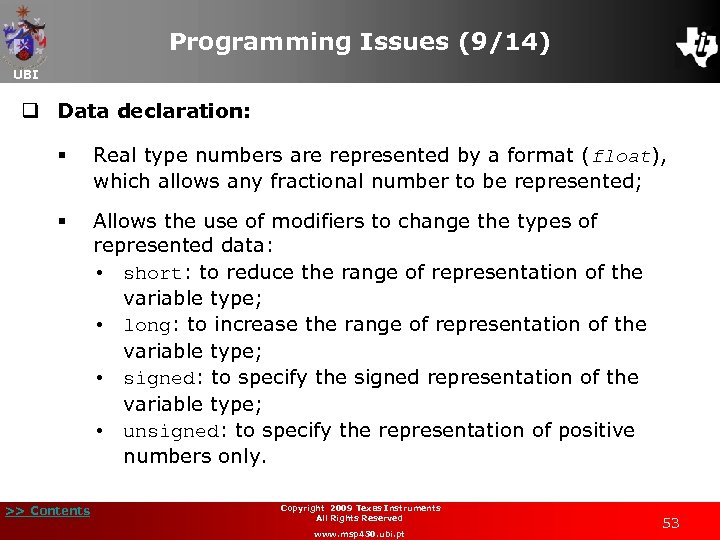Programming Issues (9/14) UBI q Data declaration: § Real type numbers are represented by a format (float), which allows any fractional number to be represented; § Allows the use of modifiers to change the types of represented data: • short: to reduce the range of representation of the variable type; • long: to increase the range of representation of the variable type; • signed: to specify the signed representation of the variable type; • unsigned: to specify the representation of positive numbers only. >> Contents Copyright 2009 Texas Instruments All Rights Reserved www. msp 430. ubi. pt 53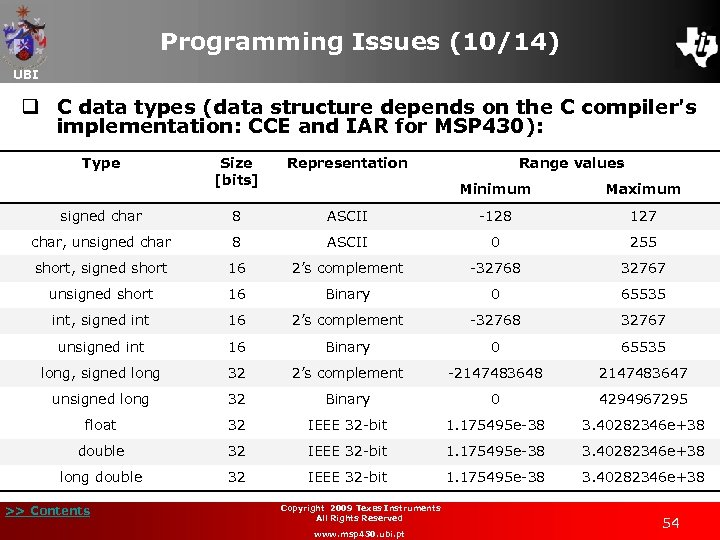Programming Issues (10/14) UBI q C data types (data structure depends on the C compiler's implementation: CCE and IAR for MSP 430): Type Size [bits] Representation signed char 8 char, unsigned char Range values Minimum Maximum ASCII -128 127 8 ASCII 0 255 short, signed short 16 2’s complement -32768 32767 unsigned short 16 Binary 0 65535 int, signed int 16 2’s complement -32768 32767 unsigned int 16 Binary 0 65535 long, signed long 32 2’s complement -2147483648 2147483647 unsigned long 32 Binary 0 4294967295 float 32 IEEE 32 -bit 1. 175495 e-38 3. 40282346 e+38 double 32 IEEE 32 -bit 1. 175495 e-38 3. 40282346 e+38 long double 32 IEEE 32 -bit 1. 175495 e-38 3. 40282346 e+38 >> Contents Copyright 2009 Texas Instruments All Rights Reserved www. msp 430. ubi. pt 54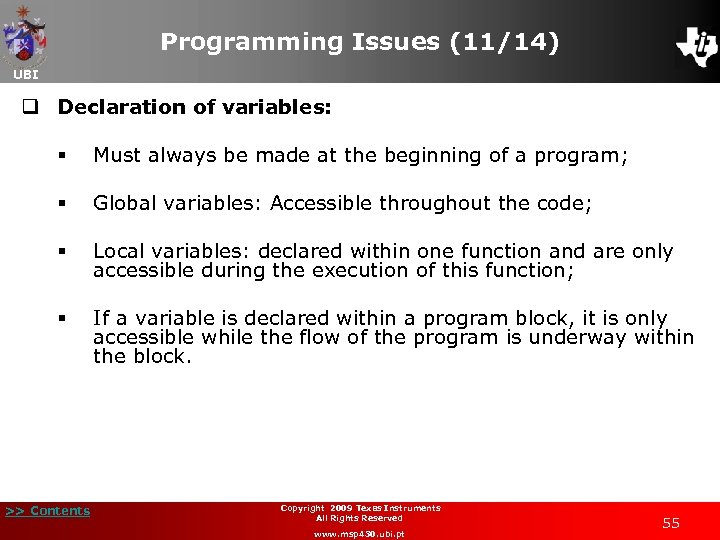Programming Issues (11/14) UBI q Declaration of variables: § Must always be made at the beginning of a program; § Global variables: Accessible throughout the code; § Local variables: declared within one function and are only accessible during the execution of this function; § If a variable is declared within a program block, it is only accessible while the flow of the program is underway within the block. >> Contents Copyright 2009 Texas Instruments All Rights Reserved www. msp 430. ubi. pt 55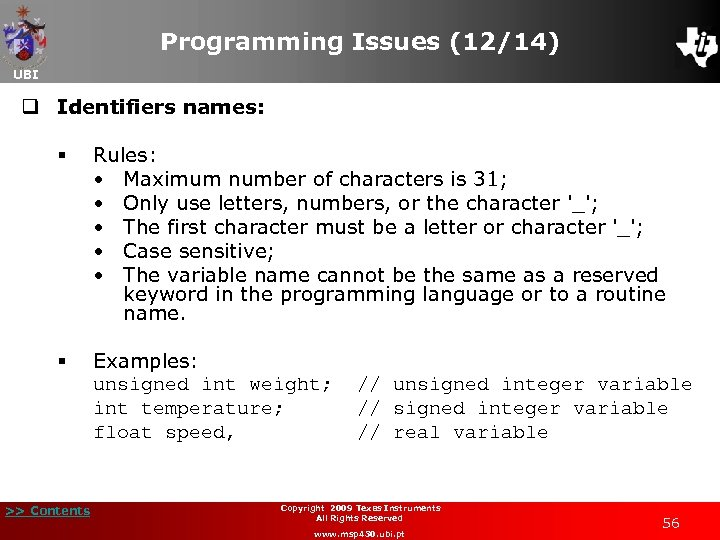Programming Issues (12/14) UBI q Identifiers names: § Rules: • Maximum number of characters is 31; • Only use letters, numbers, or the character '_'; • The first character must be a letter or character '_'; • Case sensitive; • The variable name cannot be the same as a reserved keyword in the programming language or to a routine name. § Examples: unsigned int weight; // unsigned integer variable int temperature; // signed integer variable float speed, // real variable >> Contents Copyright 2009 Texas Instruments All Rights Reserved www. msp 430. ubi. pt 56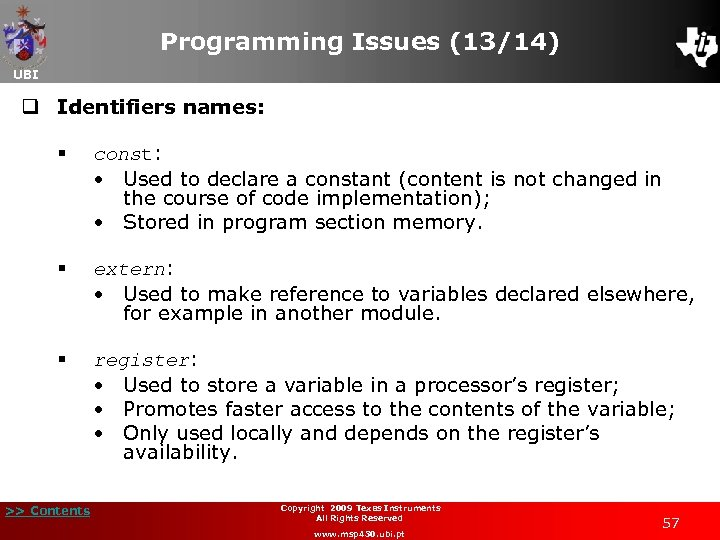Programming Issues (13/14) UBI q Identifiers names: § const: • Used to declare a constant (content is not changed in the course of code implementation); • Stored in program section memory. § extern: • Used to make reference to variables declared elsewhere, for example in another module. § register: • Used to store a variable in a processor’s register; • Promotes faster access to the contents of the variable; • Only used locally and depends on the register’s availability. >> Contents Copyright 2009 Texas Instruments All Rights Reserved www. msp 430. ubi. pt 57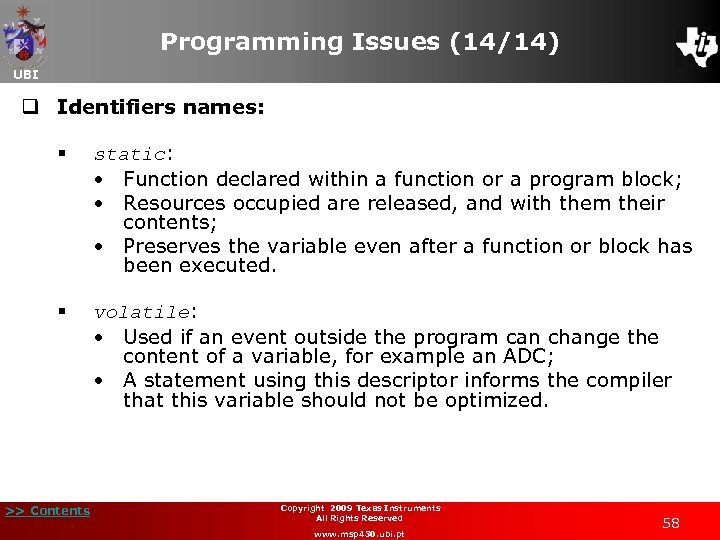Programming Issues (14/14) UBI q Identifiers names: § static: • Function declared within a function or a program block; • Resources occupied are released, and with them their contents; • Preserves the variable even after a function or block has been executed. § volatile: • Used if an event outside the program can change the content of a variable, for example an ADC; • A statement using this descriptor informs the compiler that this variable should not be optimized. >> Contents Copyright 2009 Texas Instruments All Rights Reserved www. msp 430. ubi. pt 58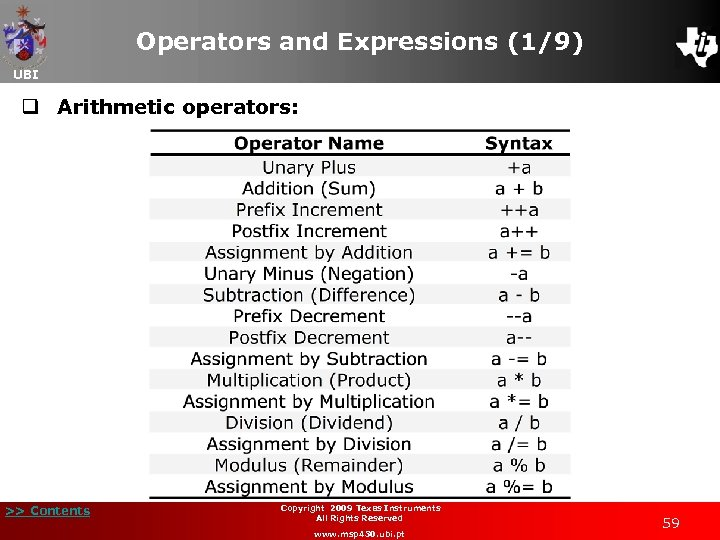Operators and Expressions (1/9) UBI q Arithmetic operators: >> Contents Copyright 2009 Texas Instruments All Rights Reserved www. msp 430. ubi. pt 59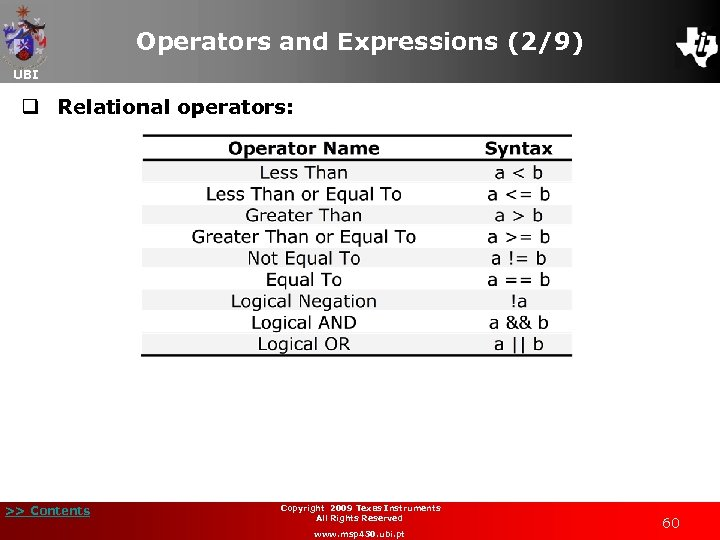Operators and Expressions (2/9) UBI q Relational operators: >> Contents Copyright 2009 Texas Instruments All Rights Reserved www. msp 430. ubi. pt 60Operators and Expressions (3/9) UBI q Other operators: >> Contents Copyright 2009 Texas Instruments All Rights Reserved www. msp 430. ubi. pt 61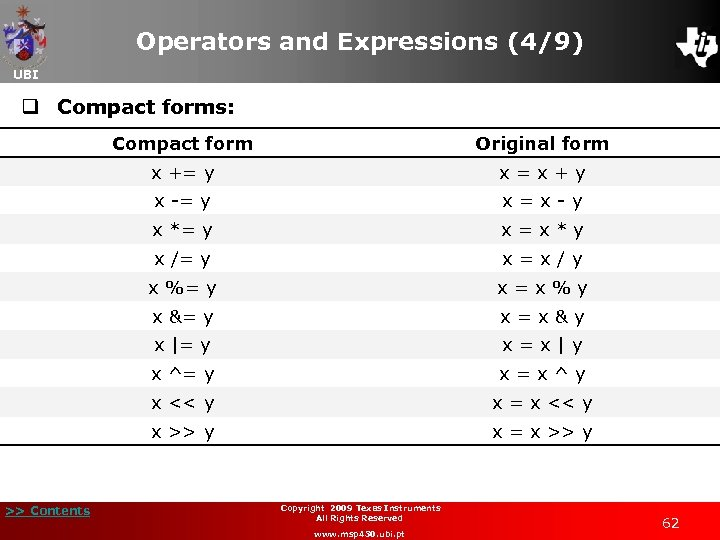Operators and Expressions (4/9) UBI q Compact forms: Compact form x += y x = x + y x -= y x = x - y x *= y x = x * y x /= y x = x / y x %= y x = x % y x &= y x = x & y x |= y x = x | y x ^= y x = x ^ y x << y x = x << y x >> y >> Contents Original form x = x >> y Copyright 2009 Texas Instruments All Rights Reserved www. msp 430. ubi. pt 62Operators and Expressions (5/9) UBI q Priority of operators: Priority Highest Lowest >> Contents Description Operator Grouping, scope, array/member access () [] -> Size of type cast (most) unary operations, … ! ~ + - & Multiplication, division, modulo * / % Addition, subtraction + Bitwise shift left and right << >> Comparisons: less-than, . . . < <= > >= Comparisons: equal and not equal == != Bitwise AND & Bitwise exclusive OR ^ Bitwise inclusive (normal) OR | Logical AND && Logical OR || Conditional expression (ternary operator) ? = += -= *= /= %= &= |= ^= <<= >>= Assignment operators Concatenation ("comma“). Copyright 2009 Texas Instruments All Rights Reserved www. msp 430. ubi. pt 63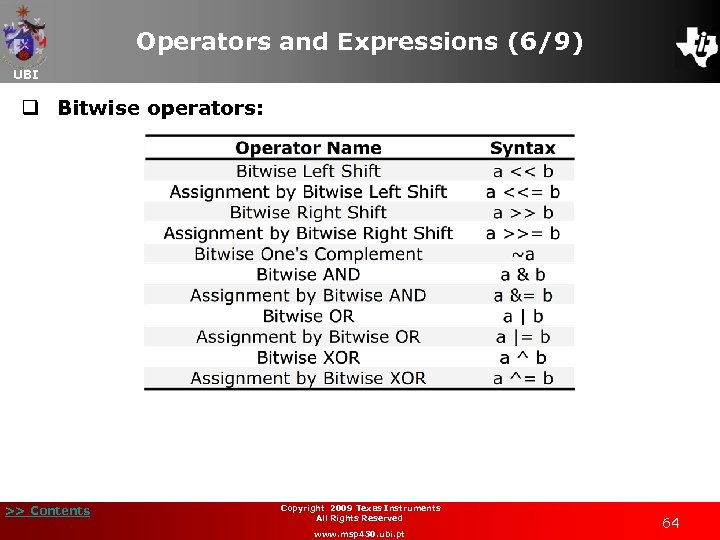Operators and Expressions (6/9) UBI q Bitwise operators: >> Contents Copyright 2009 Texas Instruments All Rights Reserved www. msp 430. ubi. pt 64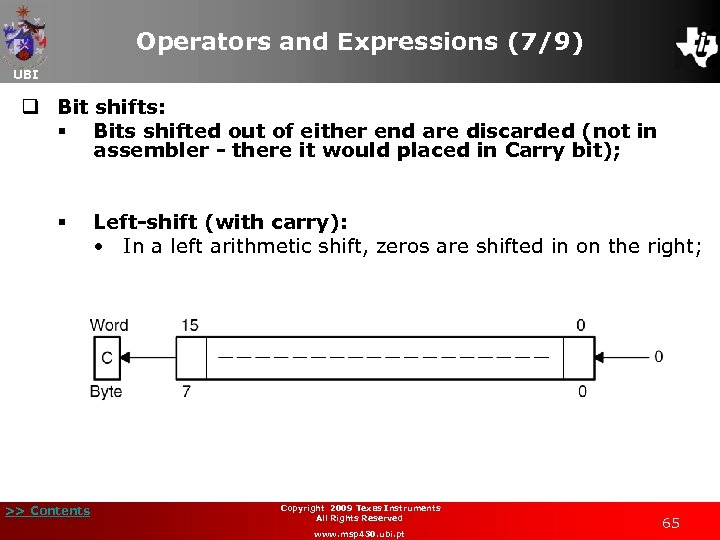Operators and Expressions (7/9) UBI q Bit shifts: § Bits shifted out of either end are discarded (not in assembler - there it would placed in Carry bit); § >> Contents Left-shift (with carry): • In a left arithmetic shift, zeros are shifted in on the right; Copyright 2009 Texas Instruments All Rights Reserved www. msp 430. ubi. pt 65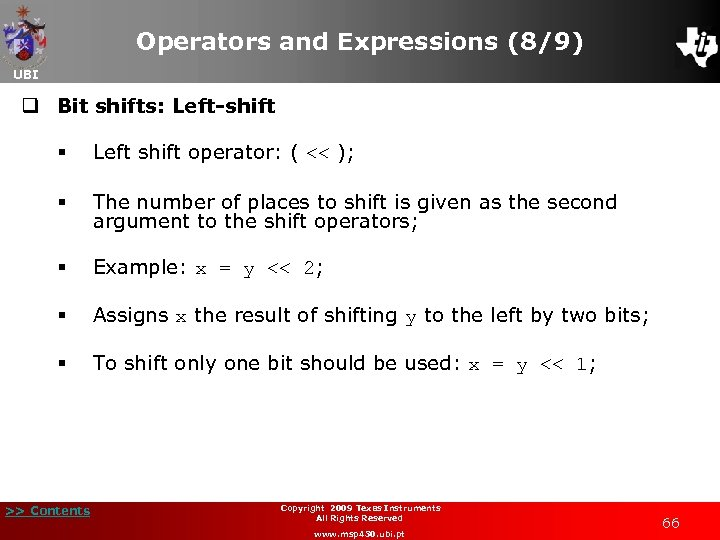Operators and Expressions (8/9) UBI q Bit shifts: Left-shift § Left shift operator: ( << ); § The number of places to shift is given as the second argument to the shift operators; § Example: x = y << 2; § Assigns x the result of shifting y to the left by two bits; § To shift only one bit should be used: x = y << 1; >> Contents Copyright 2009 Texas Instruments All Rights Reserved www. msp 430. ubi. pt 66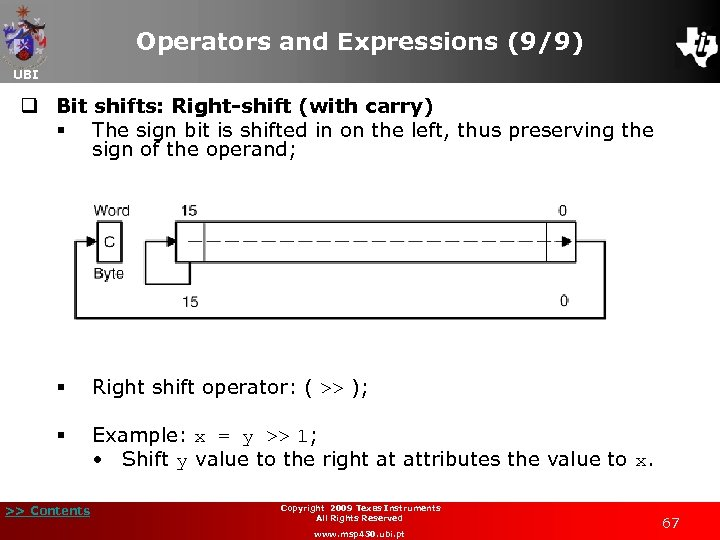Operators and Expressions (9/9) UBI q Bit shifts: Right-shift (with carry) § The sign bit is shifted in on the left, thus preserving the sign of the operand; § Right shift operator: ( >> ); § Example: x = y >> 1; • Shift y value to the right at attributes the value to x. >> Contents Copyright 2009 Texas Instruments All Rights Reserved www. msp 430. ubi. pt 67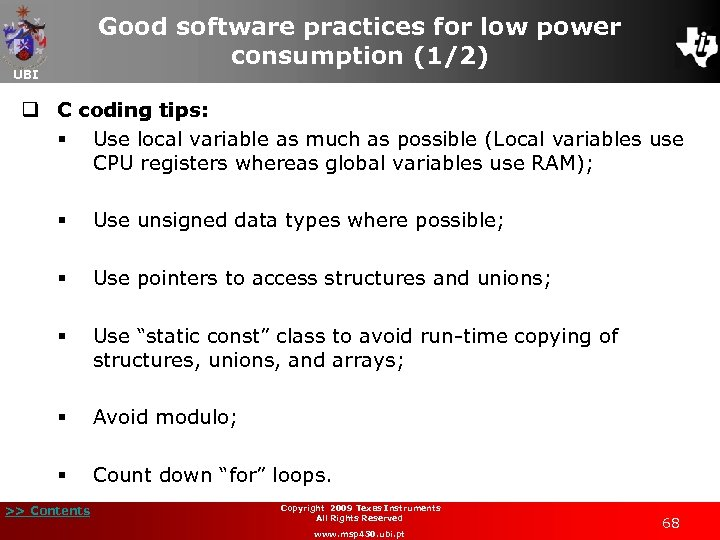Good software practices for low power consumption (1/2) UBI q C coding tips: § Use local variable as much as possible (Local variables use CPU registers whereas global variables use RAM); § Use unsigned data types where possible; § Use pointers to access structures and unions; § Use “static const” class to avoid run-time copying of structures, unions, and arrays; § Avoid modulo; § Count down “for” loops. >> Contents Copyright 2009 Texas Instruments All Rights Reserved www. msp 430. ubi. pt 68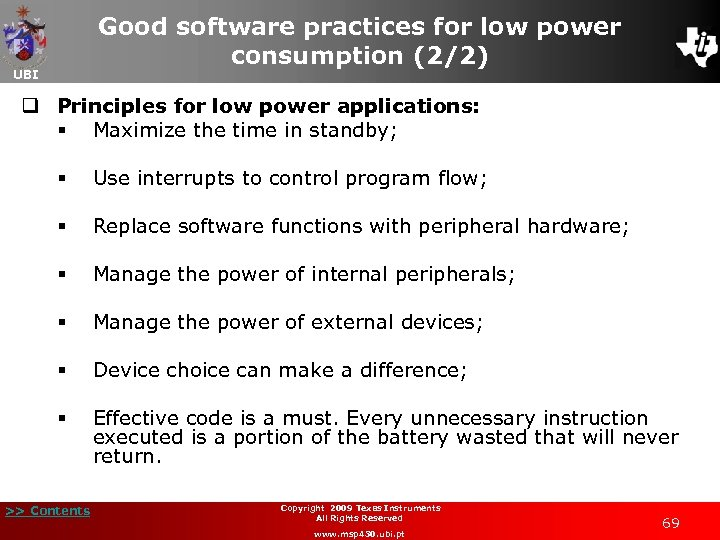Good software practices for low power consumption (2/2) UBI q Principles for low power applications: § Maximize the time in standby; § Use interrupts to control program flow; § Replace software functions with peripheral hardware; § Manage the power of internal peripherals; § Manage the power of external devices; § Device choice can make a difference; § Effective code is a must. Every unnecessary instruction executed is a portion of the battery wasted that will never return. >> Contents Copyright 2009 Texas Instruments All Rights Reserved www. msp 430. ubi. pt 69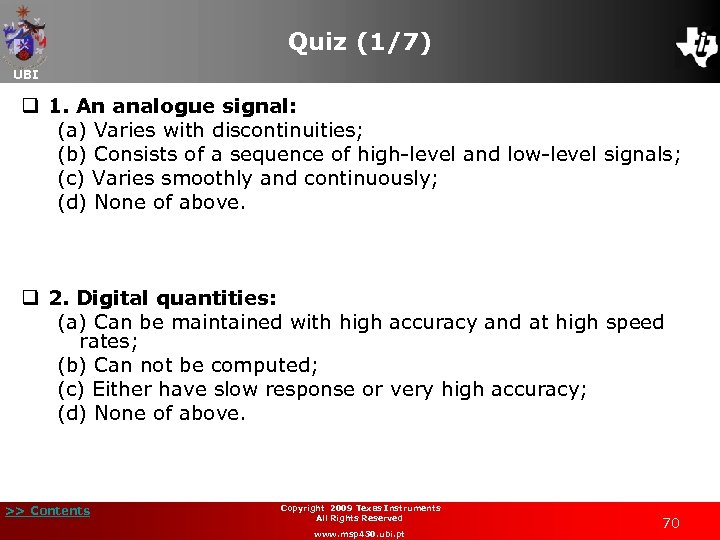Quiz (1/7) UBI q 1. An analogue signal: (a) Varies with discontinuities; (b) Consists of a sequence of high-level and low-level signals; (c) Varies smoothly and continuously; (d) None of above. q 2. Digital quantities: (a) Can be maintained with high accuracy and at high speed rates; (b) Can not be computed; (c) Either have slow response or very high accuracy; (d) None of above. >> Contents Copyright 2009 Texas Instruments All Rights Reserved www. msp 430. ubi. pt 70Quiz (2/7) UBI q 3. The highest number that can be represented by an unsigned binary 8 -bit value is: (a) 256; (b) 255; (c) 16; (d) 128. q 4. The computer performs signed arithmetic using: (a) Unsigned binary; (b) Two’s complement; (c) All of above; (d) None of above. >> Contents Copyright 2009 Texas Instruments All Rights Reserved www. msp 430. ubi. pt 71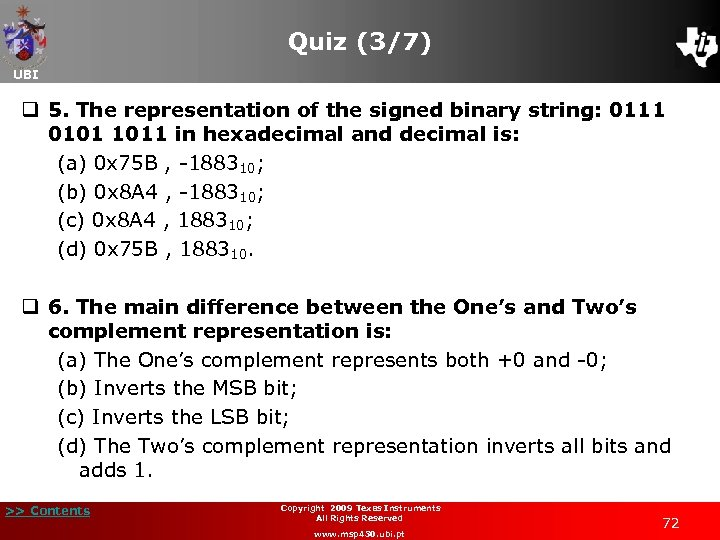Quiz (3/7) UBI q 5. The representation of the signed binary string: 0111 0101 1011 in hexadecimal and decimal is: (a) 0 x 75 B , -188310; (b) 0 x 8 A 4 , -188310; (c) 0 x 8 A 4 , 188310; (d) 0 x 75 B , 188310. q 6. The main difference between the One’s and Two’s complement representation is: (a) The One’s complement represents both +0 and -0; (b) Inverts the MSB bit; (c) Inverts the LSB bit; (d) The Two’s complement representation inverts all bits and adds 1. >> Contents Copyright 2009 Texas Instruments All Rights Reserved www. msp 430. ubi. pt 72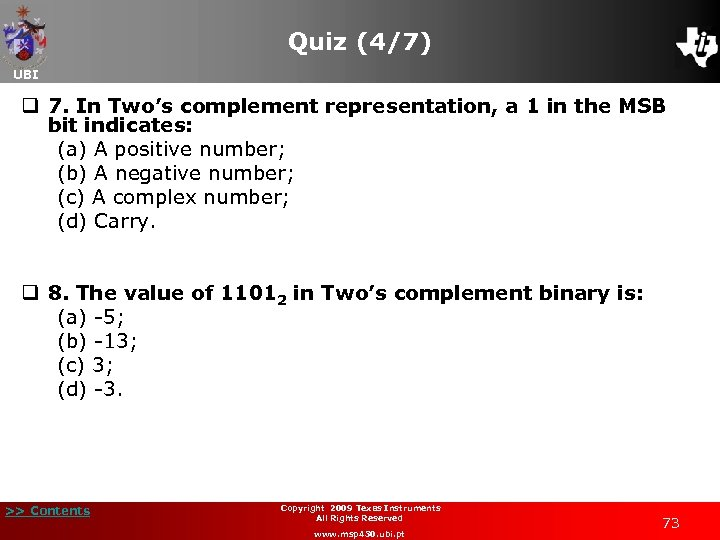Quiz (4/7) UBI q 7. In Two’s complement representation, a 1 in the MSB bit indicates: (a) A positive number; (b) A negative number; (c) A complex number; (d) Carry. q 8. The value of 11012 in Two’s complement binary is: (a) -5; (b) -13; (c) 3; (d) -3. >> Contents Copyright 2009 Texas Instruments All Rights Reserved www. msp 430. ubi. pt 73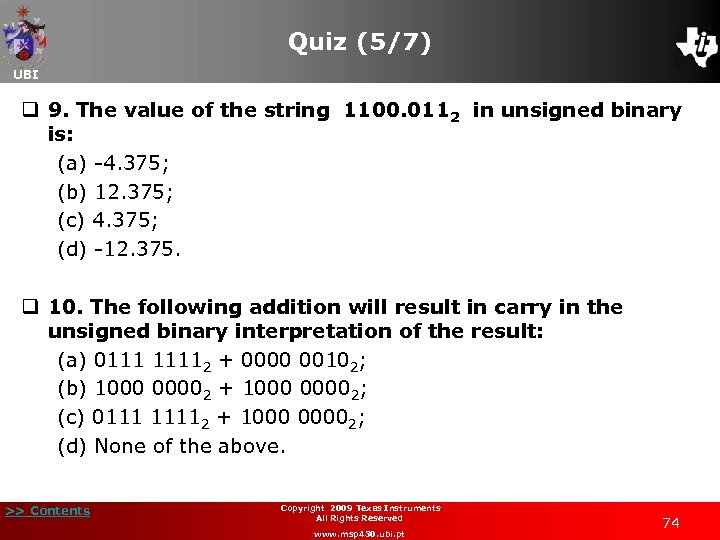Quiz (5/7) UBI q 9. The value of the string 1100. 0112 in unsigned binary is: (a) -4. 375; (b) 12. 375; (c) 4. 375; (d) -12. 375. q 10. The following addition will result in carry in the unsigned binary interpretation of the result: (a) 0111 11112 + 0000 00102; (b) 1000 00002 + 1000 00002; (c) 0111 11112 + 1000 00002; (d) None of the above. >> Contents Copyright 2009 Texas Instruments All Rights Reserved www. msp 430. ubi. pt 74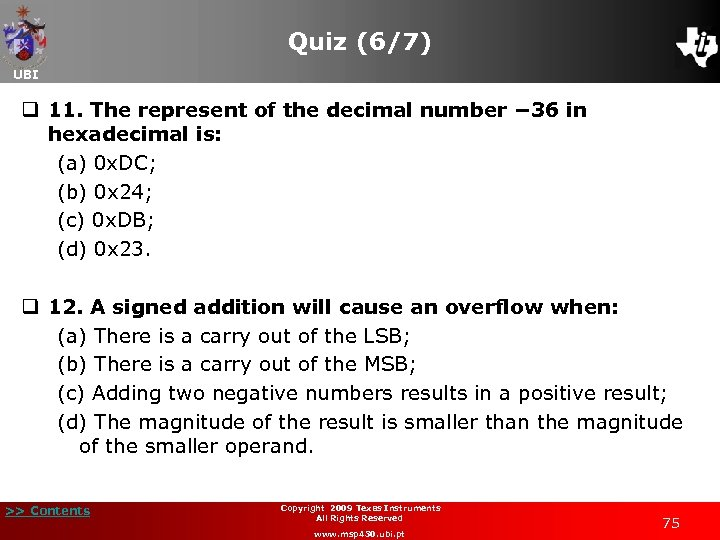Quiz (6/7) UBI q 11. The represent of the decimal number − 36 in hexadecimal is: (a) 0 x. DC; (b) 0 x 24; (c) 0 x. DB; (d) 0 x 23. q 12. A signed addition will cause an overflow when: (a) There is a carry out of the LSB; (b) There is a carry out of the MSB; (c) Adding two negative numbers results in a positive result; (d) The magnitude of the result is smaller than the magnitude of the smaller operand. >> Contents Copyright 2009 Texas Instruments All Rights Reserved www. msp 430. ubi. pt 75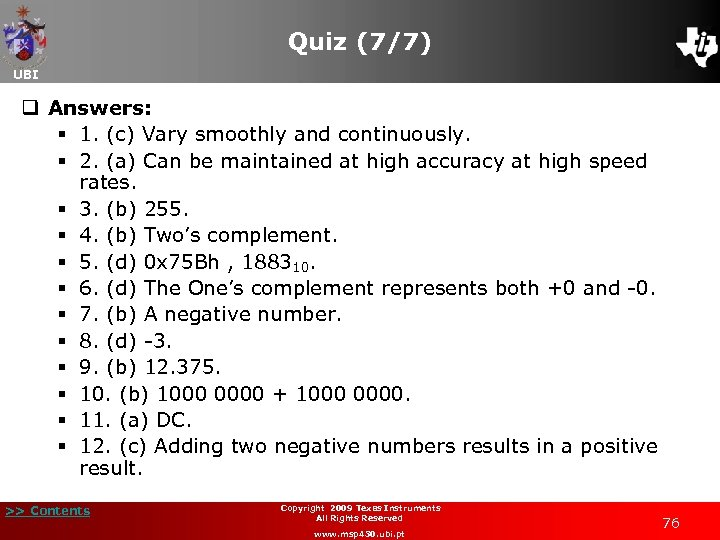Quiz (7/7) UBI q Answers: § 1. (c) Vary smoothly and continuously. § 2. (a) Can be maintained at high accuracy at high speed rates. § 3. (b) 255. § 4. (b) Two’s complement. § 5. (d) 0 x 75 Bh , 188310. § 6. (d) The One’s complement represents both +0 and -0. § 7. (b) A negative number. § 8. (d) -3. § 9. (b) 12. 375. § 10. (b) 1000 0000 + 1000 0000. § 11. (a) DC. § 12. (c) Adding two negative numbers results in a positive result. >> Contents Copyright 2009 Texas Instruments All Rights Reserved www. msp 430. ubi. pt 76